Python 与机器学习 Python & Machine Learning 2017-05-06T07:17:13.000Z http://mlblog.carefree0910.me/ 射命丸咲 Hexo “卷积神经网络”小结 http://mlblog.carefree0910.me/posts/18671318/ 2017-05-06T07:15:38.000Z 2017-05-06T07:17:13.000Z
• 相比起 NN 的全连接来说、CNN 使用了局部视野和权值共享，这使得 CNN 更适合处理结构性的数据（比如图像）
• Tensorflow 框架能帮助我们处理梯度并更新参数，这可以给实现带来极大的便利
• CNN 大体上可分为“卷积块”与“NN 块”两部分，其中卷积块为特征提取器、NN 块为“附带”的分类器
• 比起 NN 而言、CNN 的参数量会少很多，这也是许多近现代的 CNN 网络不采用全连接层而采用全局平均池化层（GAP）的原因之一
• CNN 的强大之处更多在于其提取特征的能力而非分类的能力，使用 CNN 进行特征提取后、再使用其它模型（比如 NN）进行相应的训练是一种常见的做法。事实上、这种做法有个学名叫做“迁移学习（Transfer Learning）”，感兴趣的观众老爷可以参见这里
• ]]>
<ul> <li>相比起 NN 的全连接来说、CNN 使用了局部视野和权值共享，这使得 CNN 更适合处理结构性的数据（比如图像）</li> <li>Tensorflow 框架能帮助我们处理梯度并更新参数，这可以给实现带来极大的便利</li> <li>CNN 大体上可分为“卷积块
将 NN 扩展为 CNN http://mlblog.carefree0910.me/posts/433ed5d6/ 2017-05-06T06:54:43.000Z 2017-05-06T07:15:12.000Z 往简单里说、CNN 只是多了卷积层、池化层和 FC 的 NN 而已，虽然卷积、池化对应的前向传导算法和反向传播算法的高效实现都很不平凡，但得益于 Tensorflow 的强大、我们可以在仅仅知道它们思想的前提下进行相应的实现，因为 Tensorflow 能够帮我们处理所有数学与技术上的细节

# 实现卷积层

回忆我们说过的卷积层和普通层的性质、不难发现它们的表现极其相似，区别大体上来说只在于如下三点：

• 普通层自身对数据的处理只有“激活”（$v^{\left( i \right)} = \phi_{i}\left( u^{\left( i \right)} \right)$）这一个步骤，层与层（$L_{i}$$L_{i + 1}$）之间的数据传递则是通过权值矩阵、偏置量（$w^{\left( i \right)}$$b^{\left( i \right)}$）和线性变换（$u^{\left( i + 1 \right)} = v^{\left( i \right)} \times w^{\left( i \right)} + b^{\left( i \right)}$）来完成的；卷积层自身对数据的处理则多了“卷积”这个步骤（通常来说是先卷积再激活：$v^{\left( i \right)} = \phi_{i}\left( \text{conv}\left( u^{\left( i \right)} \right) \right)$）、同时层与层之间的数据传递是直接传递的（$u^{\left( i + 1 \right)} = v^{\left( i \right)}$
• 卷积层自身多了 Kernel 这个属性并因此带来了诸如 Stride、Padding 等属性，不过与此同时、卷积层之间没有权值矩阵
• 卷积层和普通层的shape属性记录的东西不同，具体而言：
• 普通层的shape记录着上个 Layer 和该 Layer 所含神经元的个数
• 卷积层的shape记录着上个卷积层的输出和该卷积层的 Kernel 的信息（注意卷积层的上一层必定还是卷积层）

接下来就看看具体实现：

上述代码的最后几行对应着下述两个公式、这两个公式在 Tensorflow 里面有着直接对应的实现：

• 当 Padding 设置为 VALID 时，输出的高、宽分别为： 其中，符号“$\lceil\ \rceil$”代表着“向上取整”，stride 代表着步长
• 当 Padding 设置为 SAME 时，输出的高、宽分别为：

同时不难看出、上述代码其实没有把 CNN 的前向传导算法囊括进去，这是因为考虑到卷积层会利用到普通层的激活函数、所以期望能够合理复用代码。所以期望能够把上述代码定义的 ConvLayer 和前文重写的 Layer 整合在一起以成为具体用在 CNN 中的卷积层，为此我们需要利用到 Python 中一项比较高级的技术——元类（元类的介绍可以参见这里）：

在定义好基类和元类后、定义实际应用在 CNN 中的卷积层就非常简洁了。以在深度学习中应用最广泛的 ReLU 卷积层为例：

# 实现池化层

池化层比起卷积层而言要更简单一点：对于最常见的两种池化——极大池化和平均池化而言，它们所做的只是取输入的极大值和均值而已、本身并没有可以更新的参数。是故对池化层而言，我们无需维护其 Kernel、而只用定义相应的池化方法（极大、平均）即可，因此我们要求用户在调用池化层时、只提供“高”和“宽”而不提供“Kernel 个数”

注意：Kernel 个数从数值上来说与输出频道个数一致，所以对于池化层的实现而言、我们应该直接用输入频道数来赋值 Kernel 数，因为池化不会改变数据的频道数

同样的，由于 Tensorflow 已经帮助我们做好了封装、我们可以直接调用相应的函数来完成极大池化和平均池化的实现：

# 实现 CNN 中的特殊层结构

在 CNN 中同样有着 Dropout 和 Normalize 这两种特殊层结构。它们的表现和 NN 中相应特殊层结构的表现是完全一致的，区别只在于作用的对象不同

我们知道，CNN 每一层数据的维度要比 NN 中每一层数据的维度多一维：一个典型的 NN 中每一层的数据通常是$N \times p \times q$的，而 CNN 则通常是$N \times p \times q \times r$的、其中$r$是当前数据的频道数。为了让适用于 NN 的特殊层结构适配于 CNN，一个自然而合理的做法就是将$r$个频道的数据当做一个整体来处理、或说将 CNN 中$r$个频道的数据放在一起并视为 NN 中的一个神经元，这样做的话就能通过简易的封装来直接利用上我们对 NN 定义的特殊层结构。封装的过程则仍要用到元类：

# 实现 LayerFactory

我们在前三节讲述了 CNN 中卷积层、池化层和特殊层的实现，这一节我们将介绍如何定义一个简单的工厂来“生产”NN 中的层和前文介绍的这些层以方便进行应用（与上个系列中生产优化器的工厂差不多）：

以上是一些准备工作，如果由于特殊需求（比如想实验某种激活函数是否好用）实现了新的 Layer 的话、就需要更新上面对应的字典。
接下来看看核心的方法：

至此，所有 CNN 会用到的、和层结构相关的东西就已经全部实现完毕了，接下来只需在网络结构上做一些简单的更新后、CNN 的实现便能大功告成

# 扩展网络结构

将网络结构迁移到 Tensorflow 框架中并扩展出 CNN 的功能这个过程、虽然不算困难却也相当繁琐。本节将会节选出其中比较重要的部分进行说明，对于其余和上个系列实现的网络结构几乎一致的地方则不再进行注释或叙述

首先是初始化，由于我们使用的是 Tensorflow 框架、所以相应变量名的前面会一概加上“tf”两个字母：

然后我们要解决的就是上篇文章最后遗留下来的、在初始化各个权值矩阵时要把从初始化为 Numpy 数组改为初始化为 Tensorflow 数组、同时要注意兼容 CNN 的问题：

以上就是和 NN 中网络结构相比有比较大改动的地方、其余的部分则都是一些琐碎的细节。完整的代码可以参见这里，功能更为齐全、在许多细节上都进行了优化的版本则可以参见这里

]]>
<p>往简单里说、CNN 只是多了卷积层、池化层和 FC 的 NN 而已，虽然卷积、池化对应的前向传导算法和反向传播算法的高效实现都很不平凡，但得益于 Tensorflow 的强大、我们可以在仅仅知道它们思想的前提下进行相应的实现，因为 Tensorflow 能够帮我们处理所有数学与技术上的细节</p>
利用 Tensorflow 重写 NN http://mlblog.carefree0910.me/posts/24ed2586/ 2017-05-06T06:24:58.000Z 2017-05-20T01:45:52.000Z 本文将会使用 Tensorflow 框架来重写我们上个系列中实现过的 NN、观众老爷们可能会需要知道 Tensorflow 的基本知识之后才能比较顺畅地阅读接下来的内容；如果对 Tensorflow 基本不了解的话、可以先参见我写的一篇 Tensorflow 的应用式入门教程

# 重写 Layer 结构

使用 Tensorflow 来重写 NN 的流程和上个系列中我们介绍过的实现流程是差不多的，不过由于 Tensorflow 帮助我们处理了更新参数这一部分的细节，所以我们能增添许多功能、同时也能把接口写得更漂亮一些。

首先还是要来实现 NN 的基本单元——Layer 结构。鉴于 Tensorflow 能够自动获取梯度、同时考虑到要扩展出 CNN 的功能，我们需要做出如下微调：

• 对于激活函数，只用定义其原始形式、不必定义其导函数形式
• 解决上一章遗留下来的、特殊层结构的实现问题
• 要考虑当前层为 FC（全连接层）时的表现
• 让用户可以选择是否给 Layer 加偏置量

其中的第四点可能有些让人不明所以：上个系列不是刚说过、偏置量对破坏对称性是很重要的吗？为什么要让用户选择是否使用偏置量呢？这主要是因为特殊层结构中 Normalize 的特殊性会使偏置量显得冗余。具体细节会在后文讨论特殊层结构处进行说明，这里就暂时按下不表

以下是 Layer 结构基类的具体代码：

注意到我们前向传导算法中有一项“predict”参数，这主要是因为特殊层结构的训练过程和预测过程表现通常都会不一样、所以要加一个标注。该标注的具体意义会在后文进行特殊层结构 SubLayer 的相关说明时体现出来、这里暂时按下不表

在实现好基类后、就可以实现具体要用在神经网络中的 Layer 了。以 Sigmoid 激活函数对应的 Layer 为例：

得益于 Tensorflow 框架的强大（你除了这句话就没别的话说了吗……）、我们甚至连激活函数的形式都无需手写，因为它已经帮我们封装好了（事实上、绝大多数常用的激活函数在 Tensorflow 里面都有封装）

# 实现特殊层

这一节我们将介绍如何利用 Tensorflow 框架实现上个系列没有实现的特殊层结构——SubLayer，同时也会对十分常用的两种 SubLayer（Dropout、Normalize）做比上个系列深入一些的介绍

先来看看应该如何定义 SubLayer 的基类：

可以看到，得益于 Tensorflow 框架（Tensorflow 就是很厉害嘛……），本来难以处理的SubLayer 的实现变得非常简洁清晰。在实现好基类后、就可以实现具体要用在神经网络中的 SubLayer 了，先来看 Dropout：

Dropout 的详细说明自然是看原 paper 最好，这里我就大概翻译、总结一下主要内容。Dropout 的核心思想在于提高模型的泛化能力：它会在每次迭代中依概率去掉对应 Layer 的某些神经元，从而每次迭代中训练的都是一个小的神经网络。这个过程可以通过下图进行说明：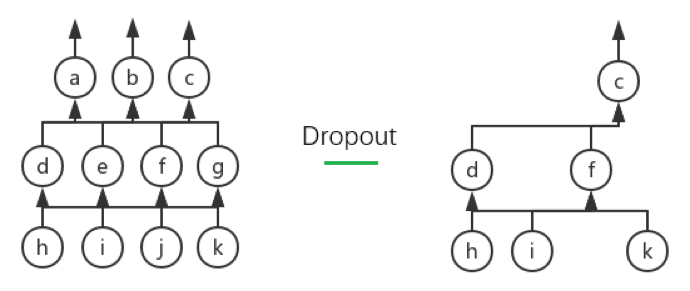上图所示的即为当drop_prob为 50%（我们所设的默认值）时、Dropout 的一种可能的表现。左图所示为原网络、右图所示的为 Dropout 后的网络，可以看到神经元 a、b、e、g、j 都被 Drop 了

Dropout 过程的合理性需要概率论上一些理论的支撑，不过鉴于 Tensorflow 框架有封装好的相应函数、我们就不深入介绍其具体的数学原理而仅仅说明其直观（以drop_prob为 50%为例，其余drop_prob的情况是同理的）：

• 在训练过程中，由于 Dropout 后留下来的神经元可以理解为“在 50%死亡概率下幸存”的神经元，所以给将它们对应的输出进行“增幅”是合理的。具体而言，假设一个神经元$n_{i}$的输出本来是$o_{i}$，那么如果 Dropout 后它被留下来了的话、其输出就应该变成$o_{i} \times \frac{1}{50\%} = 2o_{i}$（换句话说、应该让带 Dropout 的期望输出和原输出一致：对于任一个神经元$n_{i}$，设drop_prob$p$而其原输出为$o_{i}$，那么当带 Dropout 的输出为$o_{i} \times \frac{1}{p}$时、$n_{i}$的期望输出即为$p \times o_{i} \times \frac{1}{p} = o_{i}$
• 由于在训练时我们保证了神经网络的期望输出不变、所以在预测过程中我们还是应该让整个网络一起进行预测而不进行 Dropout（关于这一点，原论文似乎也表示这是一种“经试验证明行之有效”的办法而没有给出具体的、原理层面的说明）

接下来介绍一下 Normalize。Normalize 这个特殊层结构的学名叫 Batch Normalization、常简称为 BN，顾名思义，它用于对每个 Batch 对应的数据进行规范化处理。这样做的意义是直观的：对于 NN、CNN 乃至任何机器学习分类器来说，其目的可以说都是从训练样本集中学出样本在样本空间中的分布、从而可以用这个分布来预测未知数据所属的类别。如果不对每个 Batch 的数据进行任何操作的话，不难想象它们彼此对应的“极大似然分布（极大似然估计意义下的分布）”是各不相同的（因为训练集只是样本空间中的一个小抽样、而 Batch 又只是训练集的一个小抽样）；这样的话，分类器在接受每个 Batch 时都要学习一个新的分布、然后最后还要尝试从这些分布中总结出样本空间的总分布，这无疑是相当困难的。如果存在一种规范化处理方法能够使每个 Batch 的分布都贴近真实分布的话、对分类器的训练来说无疑是至关重要的

传统的做法是对输入$X$进行很久以前提到过的归一化处理、亦即：

其中$\bar{X}$表示$X$的均值、$std(X)$表示$X$的标准差（Standard Deviation）。这种做法虽然能保证输入数据的质量、但是却无法保证NN里面中间层输出数据的质量。试想NN中的第一个隐藏层$L_{2}$，它接收的输入$u^{\left( 2 \right)}$是输入层$L_{1}$的输出$v^{\left( 1 \right)} = \phi_{1}(u^{\left( 1 \right)})$和权值矩阵$w^{\left( 1 \right)}$相乘后、加上偏置量$b^{\left( 1 \right)}$后的结果；在训练过程中，虽然$v^{\left( 1 \right)}$的质量有保证，但由于$w^{\left( 1 \right)}$$b^{\left( 1 \right)}$在训练过程中会不断地被更新、所以$u^{\left( 2 \right)} = v^{\left( 1 \right)} \times w^{\left( 1 \right)} + b^{\left( 1 \right)}$的分布其实仍然不断在变。换句话说、$u^{\left( 2 \right)}$的质量其实就已经没有保证了

BN 打算解决的正是随着前向传导算法的推进、得到的数据的质量会不断变差的问题，它能通过对中间层数据进行某种规范化处理以达到类似对输入归一化处理的效果。事实上回忆上一章的内容、我们已经提到过 Normalize 的核心思想在于把父层的输出进行“归一化”了，下面我们就简单看看它具体是怎么做到这一点的

首先需要指出的是，简单地将每层得到的数据进行上述归一化操作显然是不可行的、因为这样会破坏掉每层自身学到的数据特征。设想如果某一层$L_{i}$学到了“数据基本都分布在样本空间的边缘”这一特征，这时如果强行做归一化处理并把数据都中心化的话、无疑就摈弃了$L_{i}$所学到的、可能是非常有价值的知识

为了使得中心化之后不破坏 Layer 本身学到的特征、BN 采取了一个简单却十分有效的方法：引入两个可以学习的“重构参数”以期望能够从中心化的数据重构出 Layer 本身学到的特征。具体而言：

1. 输入：某一层$L_{i}$在当前 Batch 上的输出$v^{\left( i \right)}$、增强数值稳定性所用的小值$\epsilon$
2. 过程
1. 计算当前 Batch 的均值、方差：
2. 归一化：
3. 线性变换：
3. 输出：规范化处理后的输出$y^{\left( i \right)}$

BN 的核心即在于$\gamma$$\beta$这两个参数的应用上。关于如何利用反向传播算法来更新这两个参数的数学推导会稍显繁复、我们就不展开叙述了，取而代之、我们会直接利用 Tensorflow 来进行相关的实现

需要指出的是、对于算法中均值和方差的计算其实还有一个被广泛使用的小技巧，该小技巧某种意义上可以说是用到了“动量”的思想：我们会分别维护两个储存“运行均值（Running
Mean）”和“运行方差（Running Variance）”的变量。具体而言：

1. 输入：某一层$L_{i}$在当前 Batch 上的输出$v^{\left( i \right)}$、增强数值稳定性所用的小值$\epsilon$；动量值$m$（一般取$m = 0.9$
2. 过程
首先要初始化 Running Mean、Running Variance 为 0 向量： 并初始化$\gamma$$\beta$为 1、0 向量： 然后进行如下操作：
1. 计算当前 Batch 的均值、方差：
2. 利用$\mu_{i}$$\sigma_{i}^{2}$和动量值$m$更新$\mu_{run}$$\sigma_{run}^{2}$
3. 利用$\mu_{run}$$\sigma_{run}^{2}$规范化处理输出：
4. 线性变换：
3. 输出：规范化处理后的输出$y^{\left( i \right)}$

最后提三点使用 Normalize 时需要注意的事项：

• 无论是上述的哪种算法、BN 的训练过程和预测过程的表现都是不同的。具体而言，训练过程和算法中所叙述的一致、均值和方差都是根据当前 Batch 来计算的；但测试过程中的均值和方差不能根据当前 Batch 来计算、而应该根据训练样本集的某些特征来进行计算。对于第二个算法来说，$\mu_{\text{run}}$$\sigma_{\text{run}}^{2}$天然就是很好的、可以用来当测试过程中的均值和方差的变量，对于第一个算法而言就需要额外的计算
• 对于 Normalize 这个特殊层结构来说、偏置量是一个冗余的变量；这是因为规范化操作（去均值）本身会将偏置量的影响抹去、同时 BN 本身的$\beta$参数可以说正是破坏对称性的参数，它能比较好地完成原本偏置量所做的工作
• Normalize 这个层结构是可以加在许多不同地方的（如下图所示的 A、B 和 C 处），原论文将它加在了 A 处、但其实现在很多主流的深层 CNN 结构都将它加在了 C 处；相对而言、加在 B 处的做法则会少一些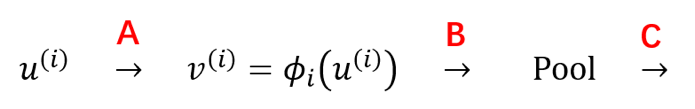在基本了解了 Normalize 对应的 BN 算法之后、我们就可以着手进行实现了：

# 重写 CostLayer 结构

在上个系列中，为了整合特殊变换函数和损失函数以更高效地计算梯度、我们花了不少代码来做繁琐的封装；不过由于 Tensorflow 中已经有了这些封装好的、数值性质更优的函数、所以 CostLayer 的实现将会变得非常简单：

短短 15 行代码就实现了上个系列中用 113 行代码才实现的所有功能，由此可窥见 Tensorflow 框架的强大

# 重写网络结构

由于 Tensorflow 重写的是算法核心部分，作为封装的网络结构其实并不用进行太大的变动；具体而言、整个网络结构需要做比较大的改动的地方只有如下两个：

• 初始化各个权值矩阵时，从初始化为 Numpy 数组改为初始化为 Tensorflow 数组、同时要注意兼容 CNN 的问题
• 不用记录所有 Layer 的激活值而只用关心输出 Layer 的输出值和 CostLayer 的损失值（在上个系列中、我们是要记录所有中间结果以进行反向传播算法的）

关于第一点我们会在后面介绍 CNN 的实现时进行说明，这里就仅看看第二点怎么做到：

注意：不难看出、get_rs是兼容 CNN 的

有了get_rs这个方法后、Tensorflow 下的网络结构的核心训练步骤就非常简洁了：

完整的、Tensorflow 版本的网络结构的代码可以参见这里，对其深入一些的介绍则在下篇文章的最后一节中进行。此外、我对 Tensorflow 提供的诸多优化器做了一个简单的封装以兼容上个系列实现的优化器的一些接口，具体的代码可以参见这里

]]>
<p>本文将会使用 Tensorflow 框架来重写我们上个系列中实现过的 NN、观众老爷们可能会需要知道 Tensorflow 的基本知识之后才能比较顺畅地阅读接下来的内容；如果对 Tensorflow 基本不了解的话、可以先参见我写的一篇 <a href="https://zhuanlan.zhihu.com/p/26645181">Tensorflow 的应用式入门教程</a></p>
从 NN 到 CNN http://mlblog.carefree0910.me/posts/7990dadf/ 2017-05-06T05:59:36.000Z 2017-05-06T07:15:08.000Z 从名字也可以看出、卷积神经网络（CNN）其实是神经网络（NN）的一种拓展，而事实上从结构上来说，朴素的 CNN 和朴素的 NN 没有任何区别（当然，引入了特殊结构的、复杂的 CNN 会和 NN 有着比较大的区别）。本文主要会说一下 CNN 的思想以及它到底在 NN 的基础上做了哪些改进，同时也会说一下 CNN 能够解决的任务类型

# “视野”的共享

CNN 的主要思想可以概括为如下两点：

• 局部连接（Sparse Connectivity）
• 权值共享（Shared Weights）

它们具有很好的直观。举一个从学术上可能不太严谨的例子：我们平时看风景时，由于视野有限、我们通常并不能将整个风景收入眼中；取而代之、我们每次只能接受视野中的、整个风景的一块“局部风景”（所谓的【局部感受野】）。如果想要欣赏整个风景的话、我们就会不断地“四处张望”。在这个过程中，我们的思想在看的过程中通常是不怎么变的；而在看完后可能整合该过程中所有视野所看到的“局部风景”并发出“这风景真美”的感慨、然后可能会根据这个感慨来调整我们的思想。在这个例子中，我们的视野就可以看作所谓的“局部连接”，我们的思想则可以看作是“共享的权值”（注：这个栗子是我开脑洞开出来的、完全不能保证其学术严谨性、还请各位观众老爷们带着批判的眼光去看待它……如果有这方面专长的观众老爷发现我完全就在瞎扯淡、还望不吝指出 ( σ’ω’)σ）

光用文字叙述可能还是有些懵懂，我来画张图（参考了一张被引用烂了的图；但由于原图有一定的误导性、所以还是打算自己画一个）（虽然很丑）：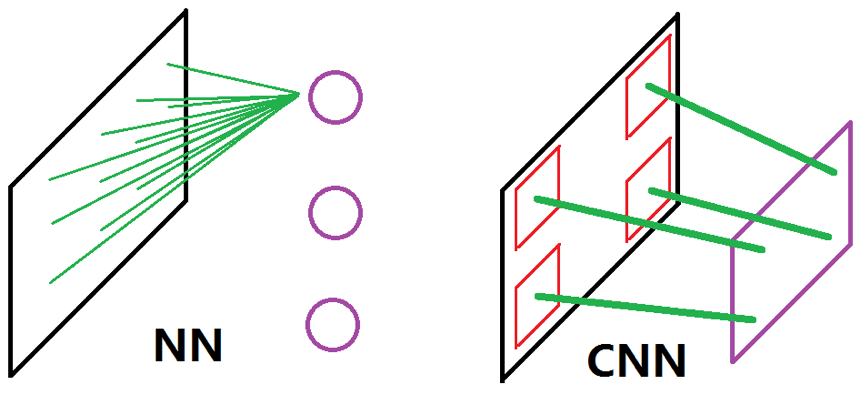这张图比较了 NN 和 CNN 的思想差别。左图为 NN，可以看到它在处理输入时是全连接的、亦即它采用的是全局感受野；同时由于各个神经元又是相对独立的、这直接导致它难以将原数据样本翻译成一个“视野”。而正如上面所说、CNN 采用的是局部感受野和共享权值，这在右图中的表现为它的神经元可以看成是“一整块”的“视野”，这块视野的每一个组成部分都是共享的权值（右图中的绿线；换句话说、右图中的四条绿线其实是同一个东西）在原数据样本的某一个局部上“看到”的东西

用上文中看风景的例子来说的话，CNN 的行为比较像一个正常人的表现、而 NN 的行为就更像是很多个能把整个风景都看在眼底的人同时看了同一个风景、然后分别感慨了一下并把这个感慨传递下去这种表现（？？？）

# 前向传导算法

CNN 的前向传导算法和上一章说明过的 NN 的前向传导算法有许多相似之处，至少从实现的层面来说它们的结构几乎一模一样。它们之间的不同之处则主要体现在如下两点：

• 接收的输入的形式不同
• 层与层之间的连接方式不同

先看第一点：对于 NN 而言、输入是一个$N \times n$的矩阵

其中$x_{1},\ldots,x_{N}$都是$n \times 1$的列向量；当输入是图像时，NN 的处理方式是将图像拉直成一个列向量。以$3 \times 3 \times 3$的图像为例（第一个 3 代指 RGB 通道，后两个 3 分别是高和宽），NN 会先把各个图像变成$27 \times 1$的列向量（亦即$n = 3 \times 3 \times 3$），然后再把它们合并、转置成一个$N \times 27$的大矩阵以当作输入

CNN 则不会这么大费周章——它会直接以原始的数据作为输入。换句话说、CNN 接收的输入是$N \times 3 \times 3 \times 3$的矩阵

可以用下图来直观认知一下该区别：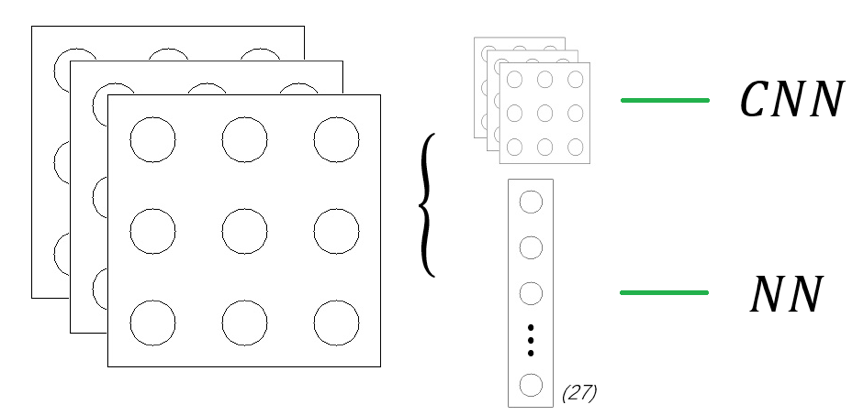所以两者的前向传导算法就可以用以下两张图来进行直观说明了：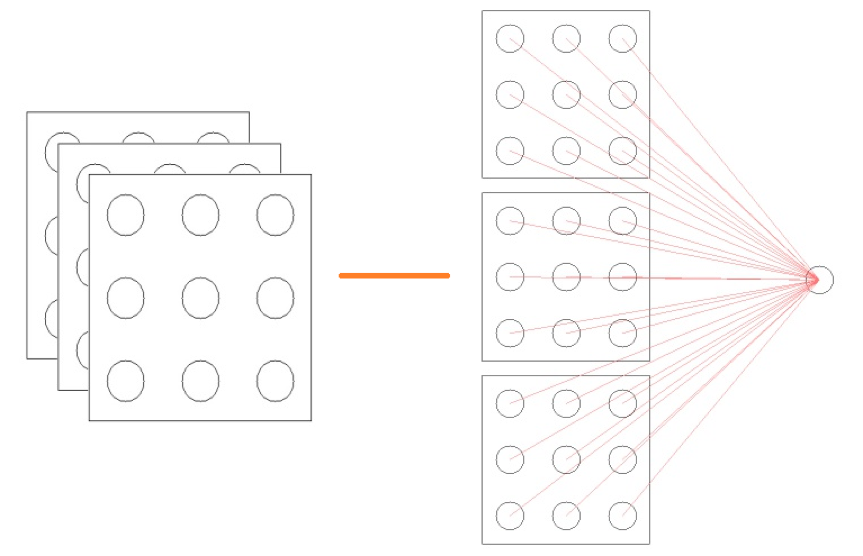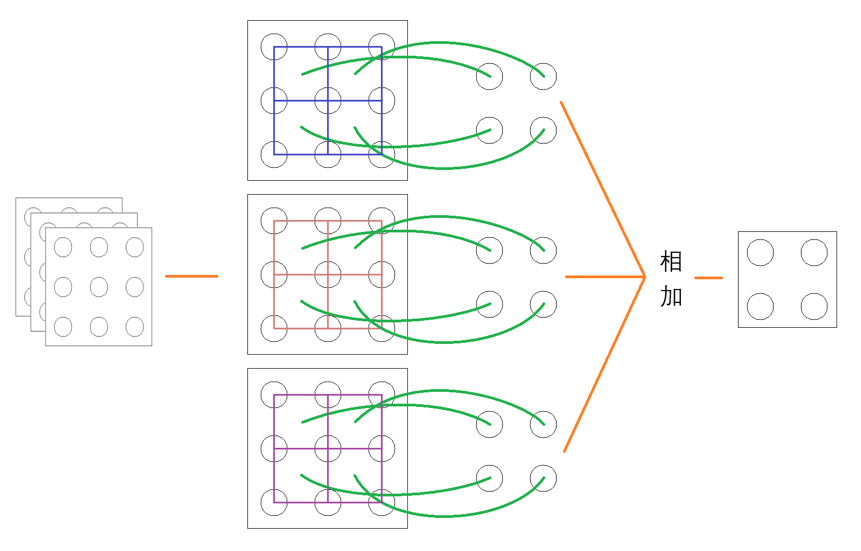（我已经尽我全力来画得好看一点了……）

下面进行进一步的说明：

• 对于一个$3 \times 3 \times 3$的输入，我们可以把它拆分成 3 个$3 \times 3$的输入的堆叠（如果把$3 \times 3 \times 3$的输入看成是一个“图像”的话，我们可以把拆分后的 3 个输入看成是该图像的 3 个“频道”；对于原始输入来讲，这 3 个频道通常就是 RGB 通道）
• 由于 NN 是全连接的，所以输入的所有信息都会直接输入给下一层的某个神经元
• 由于 CNN 是局部连接、共享权值的，一个合理的做法就是给拆分后的每个“频道”分配一个共享的“局部视野”（注意上图中三个“频道”中间都有 4 个相同颜色的正方形、且三个频道中正方形的颜色彼此不同，这就是局部共享视野的意义）（谁注意得到啊喂）。我们通常会把这三个局部视野视为一个整体并把它称作一个 Kernel 或一个 Filter
• 上面 CNN 那张图中我们用的是$2 \times 2$的局部视野，该局部视野从相应频道左上看到右上、然后看左下、最后看右下，这个过程中一共看了四次、每看一次就会生成一个输出。所以三个局部视野会分别在对应的频道上生成四个输出、亦即一个 Kernel（或说一个 Filter）会生成 3 个$2 \times 2$的输出，将它们直接相加就得到了该 Kernel 的最终输出——一个$2 \times 2$的频道

上面最后提到的“左上$\rightarrow$右上$\rightarrow$左下$\rightarrow$右下”这个“看”的过程其实就是所谓的“卷积”，这也正是卷积神经网络名字的由来。卷积本身的数学定义要比上面这个简单的描述要繁复得多，但幸运的是、实现和应用 CNN 本身并不需要具备这方面的数学理论知识（当然如果想开发更好的 CNN 结构与算法的话、是需要进行相关研究的，不过这些都已超出我们的讨论范围了）

注意：上面 CNN 的那张图中的情形为只有一个 Kernel 的情形，通常来说在实际应用中、我们会使用几十甚至几百个 Kernel 以期望网络能够学习出更好的特征——这是因为一个 Kernel 会生成一个频道，几十、几百个 Kernel 就意味着会生成几十、几百个频道，由此可以期待这大量不同的频道能够对数据进行足够强的描述（要知道原始数据可只有 3 个频道）

不难根据上文和上个系列的内容总结出 NN 和 CNN 目前为止的异同：

• NN 和 CNN 的主要结构都是层，但是 NN 的层结构是一维的（$1 \times n_{i}$）、CNN 的层结构是高维的
• NN 处理的一般是“线性”的数据，CNN 则从直观上更适合处理“结构性的”数据
• NN 层结构间会有权值矩阵作为连接的桥梁，CNN 则没有层结构之间的权值矩阵、取而代之的是层结构本身的局部视野。该局部视野会在前向传导算法中与层结构进行卷积运算来得到结果、并会直接将这个结果（或将被激活函数作用后的结果）传给下一层。因此我们常称 NN 中的层结构为“普通层”、称 CNN 中拥有局部视野的层结构为“卷积层”

可以看出、CNN 与 NN 区别之关键正在于“卷积”二字。虽然卷积的直观形式比较简单、但是它的实现却并不平凡。常用的解决方案有如下两种：

• 将卷积步骤变换成比较大规模的矩阵相乘（cs231n 里面的 stride trick 把我看哭了……）
• 利用快速傅里叶变换（Fast Fourier Transform，简称FFT）求解（只听说过，没实践过）

展开叙述它们需要用到比较深的知识、所以从略

最后介绍一下 Stride 和 Padding 的概念。Stride 可以翻译成“步长”，它描述了局部视野在频道上的“浏览速度”。设想现在有一个$5 \times 5$的频道而我们的局部视野是$2 \times 2$的，那么不同 Stride 下的表现将如下面两张图所示（只以第一排为例）：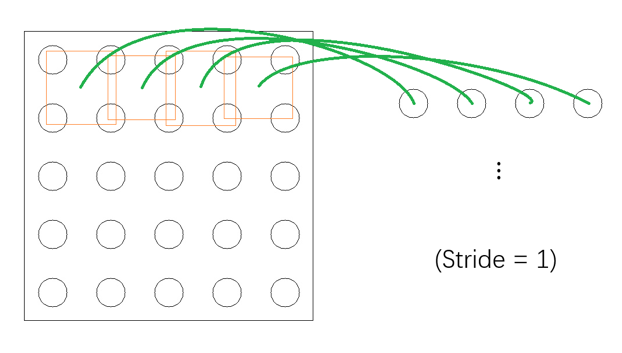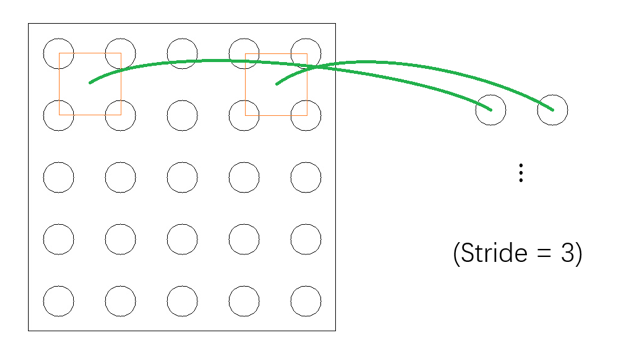（……）

可以看到上图中局部视野每次前进“一步”而下图中每次会前进“三步”

Padding 可以翻译成“填充”、其存在意义有许多种解释，一种最好理解的就是——它能保持输入和输出的频道形状一致。注意目前为止展示过的栗子中，输入频道在被卷积之后、输出的频道都会“缩小”一点。这样在经过相当有限的卷积操作后、输入就会变得过小而不适合再进行卷积，从而就会大大限制了整个网络结构的深度。Padding 正是这个问题的一种解决方案：它会在输入频道进行卷积之前、先在频道的周围“填充”上若干圈的“0”。设想现在有一个$3\times3$的频道而我们的局部视野也是$3\times3$的，如果按照之前所说的卷积来做的话、不难想象输出将会是$1\times1$的频道；不过如果我们将 Padding 设置为 1、亦即在输入的频道周围填充一圈 0 的话，那么卷积的表现将如下图所示：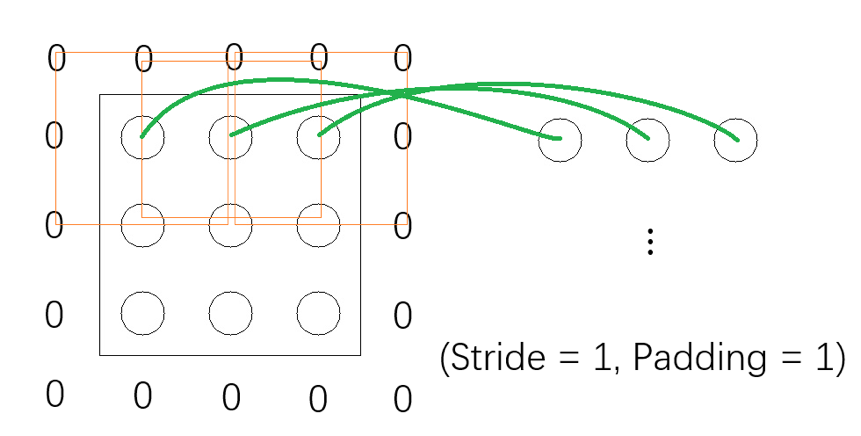可以看到当我们在输入频道外面 Pad 上一圈 0 之后、输出就变成$3 \times 3$的了，这为超深层 CNN 的搭建创造了可能性（比如有名的 ResNet）

在 cs231n 的这篇文章里面有一张很好很好很好的动图（大概位于页面中央），请允许我偷个懒不自己动手画了…… ( σ’ω’)σ

# 全连接层

全连接层是 Fully Connected Layer 的直译，常简称为 FC，它是可能会出现在 CNN 中的、一个比较特殊的结构；从名字就可以大概猜想到、FC 应该和普通层息息相关，事实上也正是如此。直观地说、FC 是连接卷积层和普通层的普通层，它将从父层（卷积层）那里得到的高维数据铺平以作为输入、进行一些非线性变换（用激活函数作用）、然后将结果输进跟在它后面的各个普通层构成的系统中：

上图中的 FC 一共有$n_{1} = 3 \times 2 \times 2 = 12$个神经元，自 FC 之后的系统其实就是上一章所介绍的 NN。换句话说、我们可以把 CNN 拆分成如下两块结构：

• 自输入开始、至 FC 终止的“卷积块”，组成卷积块的都是卷积层
• 自 FC 开始、至输出终止的“NN 块”，组成 NN 块的都是普通层

注意：值得一提的是，在许多常见的网络结构中、NN 块里都只含有 FC 这个普通层

那么为什么 CNN 会有 FC 这个结构呢？或者问得更具体一点、为什么要将总体分成卷积块和NN块两部分呢？这其实从直观上来说非常好解释：卷积块中的卷积的基本单元是局部视野，用它类比我们的眼睛的话、就是将外界信息翻译成神经信号的工具，它能将接收的输入中的各个特征提取出来；至于 NN（神经网络）块、则可以类比我们的神经网络（甚至说、类比我们的大脑），它能够利用卷积块得到的信号（特征）来做出相应的决策。概括地说、CNN 视卷积块为“眼”而视 NN 块为“脑”，眼脑结合则决策自成（？？？）。用机器学习的术语来说、则卷积块为“特征提取器”而 NN 块为“决策分类器”

而事实上，CNN 的强大之处其实正在于其卷积块强大的特征提取能力上、NN 块甚至可以说只是用于分类的一个附属品而已。我们完全可以利用 CNN 将特征提取出来后、用前面几章介绍过的决策树、支持向量机等等来进行分类这一步而无须使用 NN 块

# 池化（Pooling）

池化是 NN 中完全没有的、只属于 CNN 的特殊演算。虽然名字听上去可能有些高大上的感觉，但它的本质其实就是“对局部信息的总结”。常见的池化有如下两种：

• 极大池化（Max Pooling），它会输出接收到的所有输入中的最大值
• 平均池化（Average Pooling），它会输出接收到的所有输入的均值

池化过程其实与卷积过程类似、可以看成是局部视野对输入信息的转换，只不过卷积过程做的是卷积运算、池化过程做的是极大或平均运算而已

不过池化与卷积有一点通常是差异较大的——池化的 Stride 通常会比卷积的 Stride 要大。比如对于一个$3 \times 3$的输入频道和一个$3 \times 3$的局部视野而言：

• 卷积常常选取 Stride 和 Padding 都为 1，从而输出频道是$3 \times 3$
• 池化常常选取 Stride 为 2、Padding 为1，从而输出频道是$2 \times 2$

将 Stride 选大是符合池化的内涵的：池化是对局部信息的总结、所以自然希望池化能够将得到的信息进行某种“压缩处理”。如果将 Stride 选得比较小的话、总结出来的信息就很可能会产生“冗余”，这就违背了池化的本意

不过为什么最常见的两种池化——极大池化和平均池化确实能够压缩信息呢？这主要是因为 CNN 一般处理的都是图像数据。由经验可知、图像在像素级间隔上的差异是很小的，这就为上述两种池化提供了一定的合理性

]]>
<p>从名字也可以看出、卷积神经网络（CNN）其实是神经网络（NN）的一种拓展，而事实上从结构上来说，朴素的 CNN 和朴素的 NN 没有任何区别（当然，引入了特殊结构的、复杂的 CNN 会和 NN 有着比较大的区别）。本文主要会说一下 CNN 的思想以及它到底在 NN 的基础上做了哪些改进，同时也会说一下 CNN 能够解决的任务类型</p>
卷积神经网络综述 http://mlblog.carefree0910.me/posts/78be0d7d/ 2017-05-06T02:41:50.000Z 2017-05-06T07:15:05.000Z 卷积神经网络是 Convolutional Neural Network 的直译、常简称为 CNN，它是当今非常火热的话题——深度学习中的一种具有代表性的结构。如果提起卷积神经网络的话、许多人可能都会觉得是一个非常深奥的魔法，但如果不考虑其背后的数学理论而只想理解并应用的话、学习 CNN 其实也并不是特别困难（而且事实上相比起比较传统的机器学习算法而言、CNN 经常会由于其缺乏理论支撑而受到批评）

以下是目录：

需要特别指出的是，本系列的文章基本不会涉及任何卷积神经网络数学上的细节；一方面是因为它们相当繁复、另一方面则是因为它们也并不完全 Make Sense。卷积神经网络在因其效果拔群而大红大紫的同时、其“黑箱”程度也是非常著名的，因此我们会较多地从实现和应用层面来介绍卷积神经网络、理论方面的叙述则大多采取直观说明的方式来进行

此外，CNN 的性能分析会放在具体的应用实例（Applications）中进行，故本系列将略去这部分的内容

]]>
<p>卷积神经网络是 Convolutional Neural Network 的直译、常简称为 CNN，它是当今非常火热的话题——深度学习中的一种具有代表性的结构。如果提起卷积神经网络的话、许多人可能都会觉得是一个非常深奥的魔法，但如果不考虑其背后的数学理论而只想理解并应用的话
“神经网络”小结 http://mlblog.carefree0910.me/posts/66bacb27/ 2017-05-06T01:59:09.000Z 2017-05-06T02:00:52.000Z
• 神经网络的基本单位是层（Layer）、它是一个非常强大的多分类模型
• 神经网络的每一层（$L_{i}$）都会有一个激活函数$\phi_{i}$、它是模型的非线性扭曲力
• 神经网络通过权值矩阵$w^{\left( i \right)}$和偏置量$b^{\left( i \right)}$来连接相邻两层$L_{i}$$L_{i + 1}$，其中$w^{\left( i \right)}$能将结果从原来的维度空间线性映射到新的维度空间、$b^{\left( i \right)}$则能打破对称性
• 神经网络通过前向传导算法获取各层的激活值、通过输出层的激活值$v^{\left( m \right)}$和损失函数$L^{*}\left( x \right) = L\left( y,v^{\left( m \right)} \right)$来做决策并获得损失、通过反向传播算法算出各个 Layer 的局部梯度$\delta^{\left( i \right)}$并用各种优化器更新参数
• 合理利用一些特殊的层结构能使模型表现提升
• 当任务规模较大时、就需要考虑内存等诸多和算法无关的问题了
• ]]>
<ul> <li>神经网络的基本单位是层（Layer）、它是一个非常强大的多分类模型</li> <li>神经网络的每一层（<script type="math/tex">L_{i}</script>）都会有一个激活函数<script type="math/tex">\phi_{i
相关数学理论 http://mlblog.carefree0910.me/posts/613bbb2f/ 2017-05-06T01:38:59.000Z 2017-05-19T16:57:01.000Z 本文会叙述之前没有解决的纯数学问题，虽然它们仅会涉及到求导相关的知识、但是仍然具有一定难度

# BP 算法的推导

要想知道 BP 算法的推导，我们需要且仅需要知道两点知识：求导及其链式法则。由前文的诸多说明可知、我们至少需要知道如下几件事：

• 梯度是向量函数$f$在某点$x$上升最快的方向、其数学定义为 它是向量函数$f$对 n 个分量的偏导组成的向量。需要指出的是，我个人的习惯是在推导向量函数的梯度时，先把它分拆成单个的函数进行普通函数的求偏导计算、最后再把它们合成梯度。后文的推导也会采取这种形式
• BP 算法的初始输入是真实的类别向量$y$
• 我们的目标是让模型的输出尽可能拟合$y$。为此我们会定义：
• 预测函数$f(x)$，它是一个向量函数、会根据输入矩阵$x$输出预测向量$v^{\left( m \right)}$
• 损失函数$L^{*}\left( x \right) \triangleq L\left( y,v^{\left( m \right)} \right) = L\left( y,f\left( x \right) \right)$，它是一个标量函数、其函数值能反映$v^{\left( m \right)}$$y$的差异；差异越大、$L^{*}\left( x \right) = L\left( y,v^{\left( m \right)} \right)$的值就越大

接下来就可以进行具体的推导了。如前所述，我们会把求解梯度的过程化为若干个求解偏导数的问题、然后再把结果进行整合；换句话说，我们会先以单个的神经元为基本单元进行分析、然后再把神经元上的结果整合成 Layer 上的结果

先来通过下图来进行一些符号约定：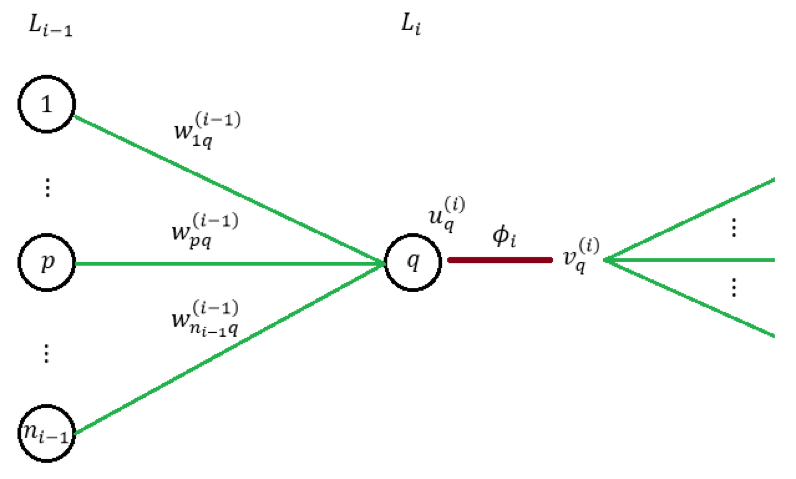其中

代表着第 k 层第 j 个神经元接收的输入；

代表着对应的激活值。注意我们在前文已经说过、局部梯度的定义可以写为

接下来我们尝试把它转化成 BP 算法中相应的公式。首先由链式法直接可得：

这就可以直接导出最朴素的 SGD 算法。继续往下推导的话会遇到两种情况：

• 当前 Layer 是 CostLayer、也就是说最后一层，此时有： 相当长的式子、里面涉及到的定义也挺多，不过其实每一步的本质都只是链式法则而已。注意最后出现了$\phi_{m}^{'}\left( u_{q}^{\left( m \right)} \right)$一项，它其实是输出层激活函数对应的导函数
• 当前 Layer 不是最后一层时，同样由链式法则可知（注意：该层的每个神经元对下一层所有神经元都会有影响） 其中 即为下一层传播回来的局部梯度；且由于 从而可知

以上就是所有的推导过程，将结果进行整合之后、不难得出前文出现过的这些公式：

• 对 CostLayer 而言、有
• 对其余 Layer 而言、有

# Softmax$+$log-likelihood 组合

这一节主要说明下常见组合——Softmax$+$log-likelihood 的梯度公式的推导，不过在此之前可能需要复习一下符号约定：

• 假设输入为$x$、输出为$y \in c_{k}$
• 假设模型在 Softmax 之前的输出为
• 假设模型的 Softmax 接受的输入为
• 假设模型在 Softmax 之后的输出为 其中
• 假设模型的损失为 log-likelihood：

接下来开始正式的推导。同样先以神经元为基本单位进行分析、可知：

注意到

所以我们只需要考虑$p = k$的情况、此时有

注意到

以及

综上所述、即得

亦即

若将 log-likelihood 改进为

即得

]]>
<p>本文会叙述之前没有解决的纯数学问题，虽然它们仅会涉及到求导相关的知识、但是仍然具有一定难度</p>
“大数据”下的网络结构 http://mlblog.carefree0910.me/posts/65c8a24f/ 2017-05-06T01:14:58.000Z 2017-05-20T01:34:39.000Z 本文标题处的“大数据”打上了引号，是因为我们所要讨论的不是当今十分火热的、真正的大数据问题、而是讨论当问题规模“相当大”时应该如何处理。我们虽然在上篇文章中实现了一个切实可用的神经网络、但它确实显得过于朴实。本文会说明如何在这个朴实模型的基础上进行拓展，这些拓展的手法不单适用于神经网络、还适用于诸多旨在解决现实生活中规模相对较大的任务的模型

# 分批（Batch）的思想

回忆上一节实现的朴素神经网络中的fit方法、可以发现每次迭代时我们都只会用整个训练集进行一次参数的更新；以 Vanilla Update 为例的话、我们进行的就是 BGD 而非 MBGD。在数据量比较大时，姑且不论 MBGD 算法和 BGD 算法本身孰优孰劣，单从内存问题来看、BGD 就不是一个可以接受的做法。因此与 MBGD 算法的思想类似、我们需要将训练集“分批（Batch）”进行训练

同样的道理，目前我们做预测时是将整个预测数据集扔给模型让它做前传算法的。当数据量比较大时、这样做显然也会引发内存不足的问题，为此我们需要分 Batch 进行前向传导并在最后做一个整合

总之在数据量变大的情况下、我们要时刻有着分 Batch 的思想。先来看看如何在训练过程中引入 Batch（以下代码需定义在fit方法中的相关位置、仅写出关键部分）：

然后是在预测过程中引入 Batch，实现的方法有两种：一种是比较常见的按个数分 Batch、一种是我们打算采用的按数据大小分 Batch。换句话说：

• 常见的做法是在每个 Batch 中放 k 个数据
• 我们的做法是在每个 Batch 中放 m 个数据、它们一共大概包含 N 个数字

其中常见做法有一个显而易见的缺点：如果单个数据很庞大的话、这样做可能还是会引发内存不足的问题。接下来就看看我们的做法相对应的具体实现：

实现完毕后、我们就能得到如下图所示的结果（以在上一篇文章最后所用的螺旋线数据集上的训练过程为例）：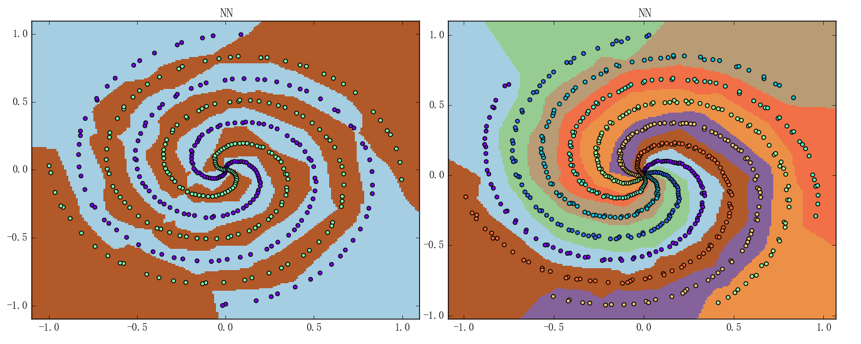其中左图的准确率为 99.0%、右图的准确率为 100%。神经网络的结构仍都是两层含 24 个神经元的 ReLU 加 Softmax$+$Cross Entropy 组合的这个结构、迭代次数仍为 1000 次、平均训练时间则分别变为 2.36秒（左图）和 3.84秒（右图）

# 交叉验证

由于针对现实任务训练出来的神经网络通常来说是很难直接进行可视化的，所以如果想要评估它的表现的话、就必须要用交叉验证。这里我们提供一种简易交叉验证的实现方法（以下代码需定义在fit方法中的相关位置、仅写出关键部分）：

仅仅简单地把数据集分开并没有意义，如果想要进行评估的话、就必须切实利用到那分出来的测试集。一种常见的做法是实时记录模型在测试集上的表现并在最后以图表的形式画出，这正是我们之前展示过的各种训练曲线的由来；要想实现这种实时记录的功能、我们需要额外地定义一些属性和方法。思路大致如下：

• 定义一个属性self._logs以存储我们的记录。该属性是一个字典、结构大致为： 其中$\text{train_log}$$\text{test_log}$为训练集和测试集的实时表现
• 常见的对模型实时表现的评估有三种：损失（cost）、准确率（acc）和 F1-score，其中前两种是通用的评估、F1-score 则针对二类分类问题（F1-score 的相关数学定义可以参见这里
• 定义三个方法，一个拿来实时记录这些评估、一个拿来输出最新的评估、一个拿来可视化评估

实现的话不难但繁、需要综合考虑许多东西并微调已有的代码；由于如果把所有变动的地方都写出来会有大量的冗余、所以这里就不写出所有细节了。感兴趣的观众老爷们可以尝试自己进行实现，我个人实现的版本则可以参见这里

实现完毕后、我们就能得到如下图所示的结果（以之前二分类螺旋线数据集上的训练过程为例）：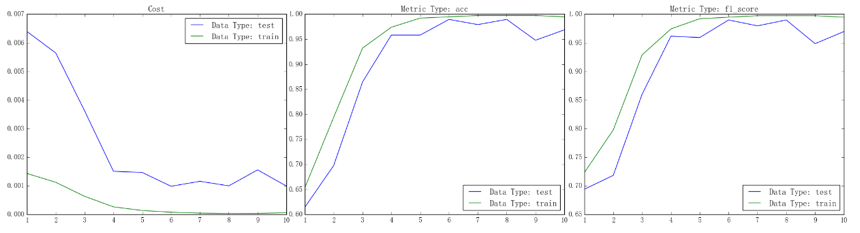从左到右依次为损失、准确率和 F1-score 的曲线，其中绿线为训练集上的表现、蓝线为测试集上的表现

# 进度条

当我们在解决现实生活中一个比较大型的问题时（比如网络爬虫或机器学习）、模型的耗时有时会达数十分钟甚至几个小时。在此期间如果程序什么都不输出的话、不免会感到些许不安：程序的运行到底到了哪个步骤？大概还需多久程序才能跑完呢？为了能在大型任务中获得即时的反馈、设计一个进度条是相当有必要的。本节拟介绍一种简单实用的进度条的实现方法，它支持记录并发程序的进度且损耗基本只来源于 Python 本身

先来看看我们的进度条是怎样的：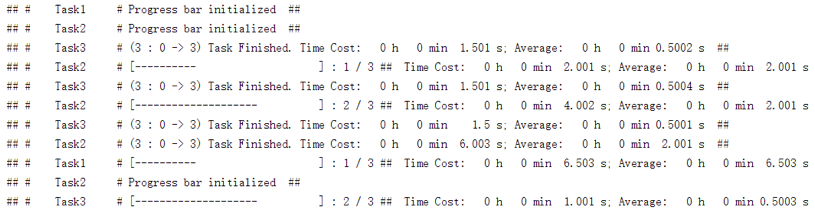其中每一行对应着一个单独任务的进度条、它有如下属性：

• 任务名字（“Test”、“Test2”和“Test3”）
• 一个形如“[- - - - - - ]”的进度显示器（紧跟在任务名字后面）
• 已完成任务数和总任务数（紧跟在进度显示器后面、以 m /n 的形式出现，其中 m 为已完成任务数、n 为总任务数）
• 总耗时和单个任务的平均耗时（紧跟在任务数后面，其中“Time Cost”后显示的是总耗时、“Average”后显示的是平均耗时，格式都是“时-分-秒”）

可以看到功能还算完备。不过虽说看上去有些复杂、但其实核心的实现只用到了time这个 Python 标准库和print这个 Python 自带的函数。总代码量虽说不算太大（110 行左右）、但有许多地方都是些琐碎的细节；所以我们这里就只说一个思路、具体的代码则可以参见这里

实现的大纲大概如下：

• 要记录任务开始时的已完成的任务数和未完成的任务数
• 要定义一个计数器，记录着总共已完成的任务数
• 要定义一个start函数和一个update函数作为初始化进度条和更新进度条的接口
• 要定义一个_flush函数来控制输出流

调用的方法也非常直观，这里举一个简单的例子：

这段代码的运行效果正如上图所示

# 计时器

对于现实生活中的任务来说，我们往往需要让模型更可控、高效；这就使得我们需要知道程序运行的各个细节、或说各个部分的时间开销。Python 有一个自带的分析程序运行开销的工具 profile、它能满足我们大部分的要求。本节拟介绍 profile 的一种更灵活的轻量级替代品——Timing 的使用，其代码量仅 60 行左右、且可以比较简单地进行各种改进、拓展（Timing 的实现会放在今后介绍 Python 装饰器时进行简要的说明，观众老爷们也可以直接参见这里

先来看一下它的效果：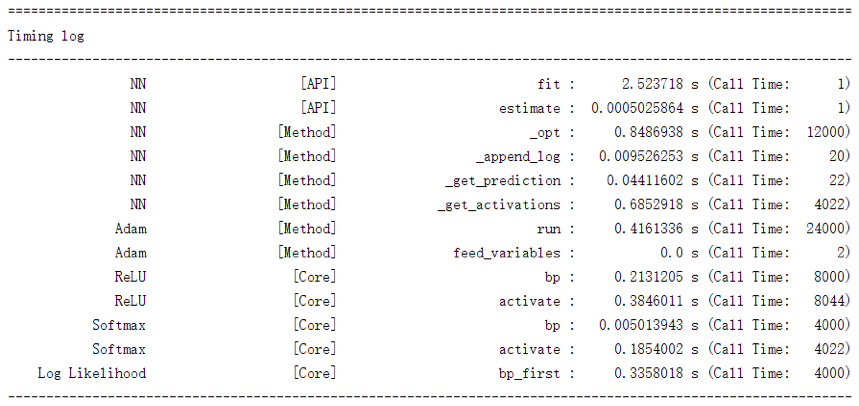该图反映的正是之前二分类螺旋线数据集上的训练过程。可以看到它将神经网络中各个组成部分的各个函数的开销情况都记录了下来、总体上来说已足够我们进行性能分析。此外、这里我们采取的是按名字排序，如有必要、完全可以定义成按总开销排序或是按平均开销排序（另外虽然我们没有记录平均开销、但是添加上平均开销这一项是平凡的）

应用 Timing 是比较简单的一件事，举一个小例子：

这段代码的运行效果如下图所示：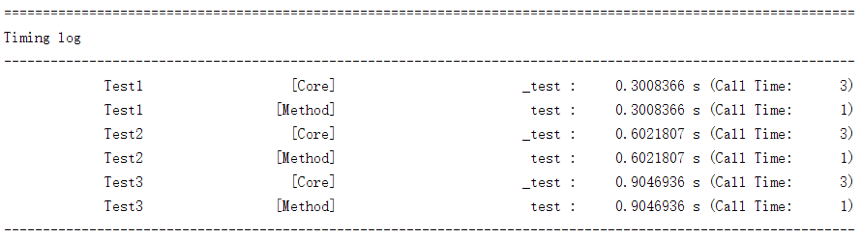]]>
<p>本文标题处的“大数据”打上了引号，是因为我们所要讨论的不是当今十分火热的、真正的大数据问题、而是讨论当问题规模“相当大”时应该如何处理。我们虽然在上篇文章中实现了一个切实可用的神经网络、但它确实显得过于朴实。本文会说明如何在这个朴实模型的基础上进行拓展，这些拓展的手法不单适用于神经网络、还适用于诸多旨在解决现实生活中规模相对较大的任务的模型</p>
朴素的网络结构 http://mlblog.carefree0910.me/posts/3bb962a6/ 2017-05-05T15:51:38.000Z 2017-05-06T02:08:37.000Z 这一节主要介绍一下如何进行最简单的封装，对于更加完善的实现则会放在下一节。由于我本人实现的最终版本有上千行，囿于篇幅、无法在这里进行叙述，感兴趣的观众老爷们可以参见这里

总结前文说明过的诸多子结构、不难得知我们用于封装它们的朴素网络结构至少需要实现如下这些功能：

• 加入一个 Layer
• 获取各个模型参数对应的优化器
• 协调各个子结构以实现前向传导算法和反向传播算法

接下来就看看具体的实现。先看其基本框架：

接下来实现加入 Layer 的功能。由于我们只打算进行朴素实现、所以应该对输入模型的 Layer 的格式做出一些限制以减少代码量。具体而言、我们对输入模型的 Layer 做出如下三个约束：

• 如果该 Layer 是第一次输入模型的 Layer 的话（亦即$L_{1}$）、则要求 Layer 的shape属性是一个二元元组，此时shape即为输入数据的维度、shape即为$L_{1}$的神经元个数$n_{1}$
• 否则（亦即$L_{i},i \geq 2$）、我们要求 Layer 输入模型时的shape属性是一元元组，其唯一的元素记录的就是该Layer的神经元个数$n_{i}$

比如说、如果我们想设计含有如下结构的神经网络：

• 含有一层 ReLU 隐藏层，该层有 24 个神经元
• 损失函数为 Sigmoid$+$Cross Entropy 的组合

那么在实现完毕后、需要能够通过如下三行代码：

来把对应的结构搭建完毕（其中 x、y 是训练集）。以下即为具体实现：

然后就需要获取各个模型参数对应的优化器并实现前向传导算法和反向传播算法了。鉴于我们实现的是朴素的版本、我们只允许用户自定义学习速率、优化器使用的算法及总的迭代次数：

这里用到了三个方法、它们的作用为：

• self._init_optimizers：根据优化器的名字、学习速率和迭代次数来初始化优化器
• self._get_activations：进行前向传导算法
• self._opt：利用局部梯度和优化器来更新模型的各个参数

它们的具体实现如下：

最后就是模型的预测了，这一部分的实现非常直观易懂：

至此、一个朴素的神经网络结构就实现完了；虽说该模型有诸多不足之处，但其基本的框架和模式却都是有普适性的、且它的表现也已经相当不错。可以通过在螺旋线数据集上做几组实验来直观地感受一下这个朴素神经网络的分类能力、结果如下图所示：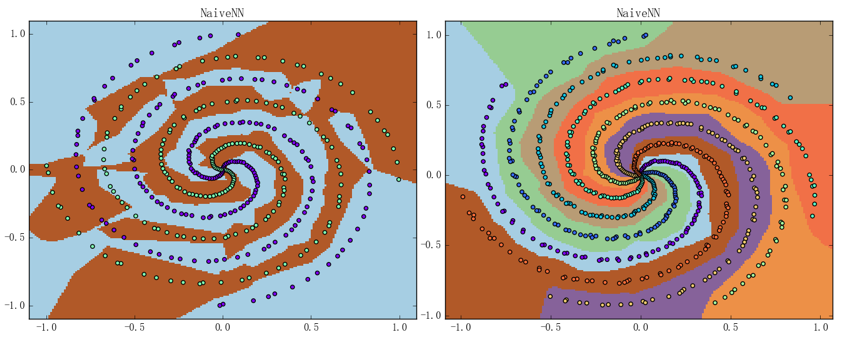左图是 4 条螺旋线的二类分类问题、准确率为 92.75%；右图为 7 条螺旋线的七类分类问题、准确率为 100%；神经网络的结构则都是两层含 24 个神经元的 ReLU 加 Softmax$+$Cross Entropy 组合的这个结构，迭代次数则为 1000 次、平均训练时间分别为 0.74秒（左图）和 1.04秒（右图）。注意到虽然我们使用的螺旋线数据集的“旋转程度”比之前使用过的螺旋线数据集的都要大不少、但是神经网络的表现仍然相当不错

]]>
<p>这一节主要介绍一下如何进行最简单的封装，对于更加完善的实现则会放在下一节。由于我本人实现的最终版本有上千行，囿于篇幅、无法在这里进行叙述，感兴趣的观众老爷们可以参见<a href="https://github.com/carefree0910/MachineLearning/blob/master/NN/Basic/Networks.py">这里</a></p> <p>总结前文说明过的诸多子结构、不难得知我们用于封装它们的朴素网络结构至少需要实现如下这些功能：</p> <ul> <li>加入一个 Layer</li> <li>获取各个模型参数对应的优化器</li> <li>协调各个子结构以实现前向传导算法和反向传播算法</li> </ul>
参数的更新 http://mlblog.carefree0910.me/posts/55a23cf0/ 2017-05-05T15:06:13.000Z 2017-05-06T02:32:29.000Z 我们之前曾简单地描述过如何使用随机梯度下降来更新参数，本文则主要会介绍一些应用得更多、效果更好的算法。正如上个系列最后所提及的，这些梯度下降的拓展算法从思想上来说和梯度下降法类似、区别则可以简练地概括如下两点：

• 更新方向不是简单地取为梯度
• 学习速率不是简单地取为常值

虽然我们不会深入地叙述这些算法背后复杂的数学基础、但我们会对每种算法都提供一些直观的解释。需要指出的是、这些算法都是利用局部梯度来获得一个更好的“梯度”、从而使得“梯度下降”变得更优

具体而言、原始的梯度为：

若想把它向量化、就不得不考虑上训练集中的样本数$N$，此时：

• 权值矩阵：$w^{\left( i - 1 \right)}$的维度为$n_{i - 1} \times n_{i}$
• 输出向量：$v^{\left( i - 1 \right)}$的维度为$N \times n_{i - 1}$
• 局部梯度：$\delta^{\left( i \right)}$的维度为$N \times n_{i}$

且有

换句话说、原始梯度的向量化形式即为：

而本节所要说明的诸多算法、大多都是利用$\Delta w^{\left( i - 1 \right)}$和其它属性来得到一个比$\Delta w^{\left( i - 1 \right)}$更好的“梯度”$\Delta^{*}w^{\left( i - 1 \right)}$、进而把梯度下降从

变成

在接下来的讨论中，我们统一使用$w$代指要更新的参数、用$\Delta w_{t}$$\Delta^{*}w_{t}$代指第 t 步迭代中得到的原始梯度和优化后的梯度、用$\eta$代指学习速率。首先需要指出的是，在众多深度学习的成熟框架中、参数的更新过程常常会被单独抽象成若干个模型，我们常常会称这些模型为“优化器（Optimizer）”。顾名思义、优化器能够根据模型的参数和损失来“优化”模型；具体而言，优化器至少需要能够利用各种算法并根据输入的参数与对应的梯度来进行参数的更新。对于有自身 Graph 结构的深度学习框架而言（比如 Tensorflow），用户甚至只需将参数更新的算法和最终的损失值提供给其优化器、然后该优化器就能够利用 Graph 结构来自动更新各个部分的参数

我们所打算实现的优化器属于最朴素的优化器——根据算法与梯度来更新相应参数；由后文的讨论可知，比较优秀的算法在每一步迭代中计算梯度时都不是独立的、而会利用上以前的计算结果。综上所述、可知优化器的框架应该包括如下三个方法：

• 接收欲更新的参数并进行相应处理的方法
• 利用梯度和自身属性来更新参数的方法
• 在完成参数更新后更新自身属性的方法

尽管一个朴素优化器的实现比较平凡，但对于帮助我们理解各种算法而言还是足够的。考虑到不同算法对应的优化器有许多行为一致的地方，为了合理重复利用代码、我们需要把它们的共性所对应的实现抽象出来：

接下来就看看各种常用的参数更新算法的说明和相应实现

# Vanilla Update

Vanilla 在机器学习中常用来表示“朴实的”、“平凡的”，换句话说、Vanilla
Update 和最普通的梯度下降法别无二致，亦即：

在实际实现中、Vanilla Update 通常以小批量梯度下降法（MBGD）的形式出现：

其中$dw$通常会是一个矩阵（对应 MBGD 算法）而非一个数（对应 SGD 算法）。

注意：即使是 SGD、其实也属于 Vanilla Update

# Momentum Update

Vanilla Update 的缺点是比较明显的：以 MBGD 为例，它每一步迭代中参数的更新是完全独立的、亦即第t步参数的更新方向只依赖于当前所用的 batch，这在物理意义上是不太符合直观的。可以进行如下设想：

• 将损失函数的图像想象成一个山谷、我们的目的是达到谷底
• 将损失函数某一点的梯度想象成该点对应的坡度
• 将学习速率想象成沿坡度行走的速度

如果是 Vanilla Update 的话，就相当于可能会出现明明前一秒还在以很快的速度往左走、这一秒就突然开始以很快的速度往右走。这种“行进模式”之所以违背直观、是因为没有考虑到我们都很熟悉的“惯性”。Momentum Update 正是通过尝试模拟物体运动时的“惯性”以期望增加算法收敛的速度和稳定性，其优化公式为：

其中梯度$\Delta w_{t}$的物理意义即为“动力”、$v_{t}$的物理意义即为第 t 步迭代中参数的“行进速度”、$\rho$的物理意义即为惯性，它描述了上一步的行进速度会在多大程度上影响到这一步的行进速度。易知当$\rho = 0$时、Momentum Update等价于 Vanilla Update

一般来说我们不会把$\rho$设置为一个常量、而会把它设置成一个会随训练过程的推进而变动的变量；同时一般来说、我们会将$\rho$的初始值设为 0.5 并逐步将它加大至 0.99。该做法蕴含着如下两个思想：

• 认为训练刚开始时的梯度会比较大而训练后期时梯度会变小，通过逐步调大$\rho$、我们能够使更新的步伐一直保持在比较大的水平
• 认为当我们接近谷底时、我们应该尽量减少“动力”带来的影响而保持原有的方向前进。这是因为如果每一步都直接往谷底方向走（亦即运动仅受动力影响）的话、就会很容易由于动力大小难以拿捏而引发震荡

该做法所对应的实现如下：

当然也不是说只能用这种方法来调整$\rho$的值，对于一些特殊的情况、确实是会有更好且更具针对性的更新策略的

# Nesterov Momentum Update

从名字不难想象，Nesterov Momentum Update 方法是基于 Momentum Update 方法的，它由 Ilya Sutskever 在 Nesterov 相关工作（Nesterov Accelerated Gradient，常简称为 NAG）的启发下提出。它在凸优化问题下的收敛性会比传统的 Momentum Update 要更好，而在实际任务中它也确实经常表现得更优

Nesterov Momentum Update 的核心思想在于想让算法具有“前瞻性”。简单来说、它会利用“下一步”的梯度而不是“这一步”的梯度来合成出最终的更新步伐（所谓更新步伐、可以直观地理解为“更新方向$\times$更新幅度”）。可以通过下图来直观地认知这个过程：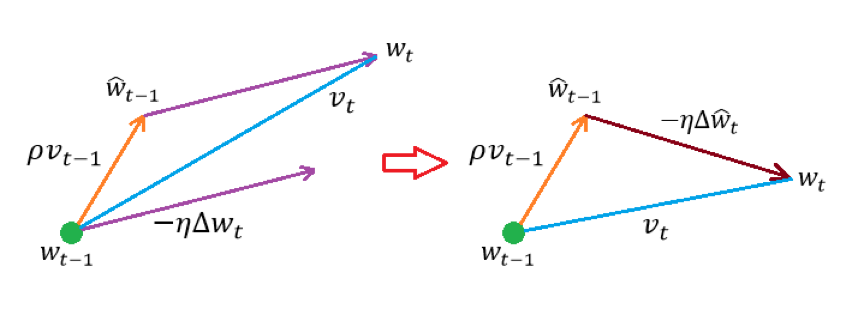左图为普通的 Momentum Update、$v_{t}$经由如下两部分合成而得：

• 起点$w_{t - 1}$处的行进速度$\rho v_{t - 1}$
• 中继点${\hat{w}}_{t - 1}$处的更新步伐$- \eta\Delta w_{t}$$w_{t - 1}$处的负梯度与学习速率的乘积）

右图则为 Nesterov Momentum Update、$v_{t}$经由如下两部分合成而得：

• 起点$w_{t - 1}$处的行进速度$\rho v_{t - 1}$
• 中继点${\hat{w}}_{t - 1}$处的更新步伐$- \eta\Delta{\hat{w}}_{t}$${\hat{w}}_{t - 1}$处的负梯度与学习速率的乘积）

于是不难写出 Nesterov Momentum Update 的优化公式：

但是这里$\Delta{\hat{w}}_{t}$的计算却不是一个平凡的问题。对此、Yoshua Bengio 等人在论文《Advances In Optimizing Recurrent Networks》里面提出了一个利用到换参法的解决方案。具体而言、令：

注意到

从而

综上所述、不难得到换参后的优化公式：

可以看出该更新公式和 Momentum Update 中的更新公式非常类似、从而在实现层面上也基本相同。事实上、只需将 Momentum 优化器中的run方法改写为：

然后再让 Nesterov Momentum Update 对应的优化器（NAG 优化器）继承 Momentum 优化器、并把self._is_nesterov这项属性设为 True 即可：

# RMSProp

RMSProp 方法与 Momentum 系的方法最根本的不同在于：Momentum 系算法是通过搜索更优的更新方向来进行优化、而 RMSProp 则是通过实时调整学习速率来进行优化。具体而言、它的优化公式为：

其中有两个变量是需要注意的：

• 中间变量$\nabla^{2}$，它是从算法开始到当前步骤的所有梯度的某种“累积”
• 衰减系数$\rho$，它反映了比较早的梯度对当前梯度的影响、$\rho$越小则影响越小

换句话说、在 RMSProp 算法中，“累积”的梯度越小会导致当前更新步伐越大、反之则会越小。关于这种做法的合理性有许多种解释，我可以提供一个仅供参考的说法：如果徘徊回了原点自然需要奋发图强地开辟新天地、如果已经走了很远自然应该谨小慎微（？？？）

从直观上来说、Adam 算法很像是 Momentum 系算法和 RMSProp 算法的结合（中间变量$\Delta$的相关计算类似于 Momentum 系算法对更新方向的选取、中间变量$\nabla$的相关计算则类似于 RMSProp 算法对学习速率的调整）。同样的、我们跳过其背后的那一套数学理论并仅说明如何进行实现：

# Factory

前 5 小节分别介绍了 5 种常用的优化算法及对应的优化器的实现、这一小节主要介绍的就是如何应用这些实现好的优化器。虽说直接对它们进行调用也无不可，但是考虑到编程中的一些“套路”、我们可以实现一个简单的工厂来“生产”这些优化器：

至此、我们就对如何更新神经网络中的参数进行了比较全面的说明；结合上一节所实现的 Layer 结构、我们接下来要做的事情就很明确了：定义一个总的框架、把 Layer、Optimizer 有机地结合在一起、从而得到最终能用的 NN 模型

]]>
<p>我们之前曾简单地描述过如何使用随机梯度下降来更新参数，本文则主要会介绍一些应用得更多、效果更好的算法。正如上个系列最后所提及的，这些梯度下降的拓展算法从思想上来说和梯度下降法类似、区别则可以简练地概括如下两点：</p> <ul> <li>更新方向不是简单地取为梯度</li> <li>学习速率不是简单地取为常值</li> </ul> <p>虽然我们不会深入地叙述这些算法背后复杂的数学基础、但我们会对每种算法都提供一些直观的解释。需要指出的是、这些算法都是利用局部梯度来获得一个更好的“梯度”、从而使得“梯度下降”变得更优</p>
特殊的层结构 http://mlblog.carefree0910.me/posts/a33ff165/ 2017-05-05T14:47:43.000Z 2017-05-06T02:25:34.000Z 在神经网络模型中有一类特殊的 Layer 结构——它们不会独立地存在、而会“依附”在某个 Layer 之后以实现某种特定的功能。一般我们会称这种特殊的 Layer 结构为附加层（SubLayer）

CostLayer 算是一个比较特殊的 SubLayer：它附加在输出层的后面、能够根据输出进行相应的变换并得到模型的损失。“根据输出得到损失”即是 CostLayer 实现的特定的功能。对于一般的 SubLayer、它的思想是清晰的：为了在 Layer 的输出的基础上进行一些变换以得到更好的输出；换句话说、SubLayer 通常可以优化 Layer 的输出

对于 SubLayer 和 SubLayer、SubLayer 和 Layer 之间的关系，我们可以类比于决策树中的根节点（Root）、叶节点（Leaf）等概念来提出“根层（Root Layer）”和“叶层（Leaf Layer）”的概念。不妨以下图为例：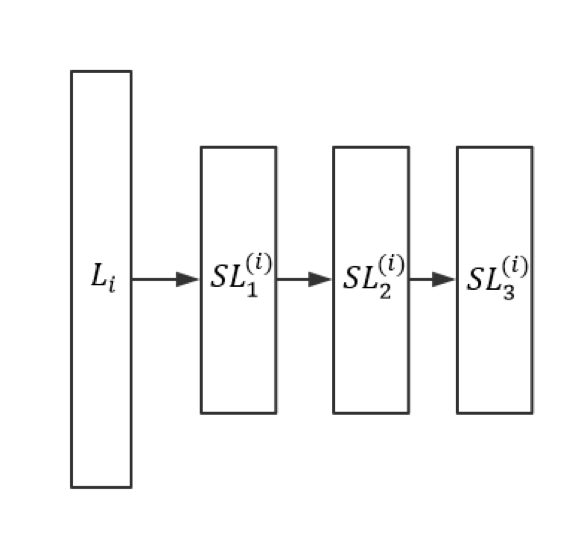其中$L_{i}$为第 i 层 Layer、$SL_{1}^{\left( i \right)},SL_{2}^{\left( i \right)},SL_{3}^{\left( i \right)}$为附加在$L_{i}$后的三个 SubLayer，且：

• $L_{i},SL_{1}^{\left( i \right)},SL_{2}^{\left( i \right)}$分别为$SL_{1}^{\left( i \right)},SL_{2}^{\left( i \right)},SL_{3}^{\left( i \right)}$的父层
• $SL_{1}^{\left( i \right)},SL_{2}^{\left( i \right)},SL_{3}^{\left( i \right)}$分别为$L_{i},SL_{1}^{\left( i \right)},SL_{2}^{\left( i \right)}$的子层
• $L_{i}$$SL_{1}^{\left( i \right)},SL_{2}^{\left( i \right)},SL_{3}^{\left( i \right)}$的 Root Layer
• $SL_{3}^{\left( i \right)}$$L_{i}$的 Leaf Layer

从 SubLayer 的思想可以看出、SubLayer 很像一个“局部优化器”；不过和下一节中要介绍的优化器不同，它不是通过更新模型参数来优化模型、而是通过变换 Layer 的输出来优化模型

在进一步叙述之前、我们需要先定义一下层结构之间的“关联”是什么。具体而言：

• Layer 和 Layer 之间的关联即为相应的权值矩阵，比如$L_{i},L_{i + 1}$之间的关联即为$w^{\left( i \right)}$
• SubLayer 之间的关联亦即 SubLayer 和 Root Layer 之间的关联都只是“占位符”、它们没有任何实际的作用。这其实是符合 SubLayer 作为“局部优化器”的定位的

从而 SubLayer 的所有行为大体上可以概括如下：

• 在前向传导中、它会根据自身的属性和算法来优化从父层处得到的更新
• 在反向传播中、它会有如下三种行为：
• SubLayer 之间的关联以及 SubLayer 和 Root Layer 之间的关联不会被更新、因为它们仅仅是占位符
• SubLayer 作为“局部优化器”、本身可能会有一些参数，这些参数则可能会被 BP 算法更新、但影响域仅在该 SubLayer 的内部（Normalize 会是一个很好的例子）
• Layer 之间的关联的更新是通过 Leaf Layer 完成的。具体而言、$L_{i}$的 Leaf Layer 会利用$L_{i}$的激活函数来完成局部梯度的计算

最后这里所谓的“利用 Leaf Layer”可以通过下面两张图来直观认知在存在 SubLayer 的情况下、前向传导算法和反向传播算法的表现：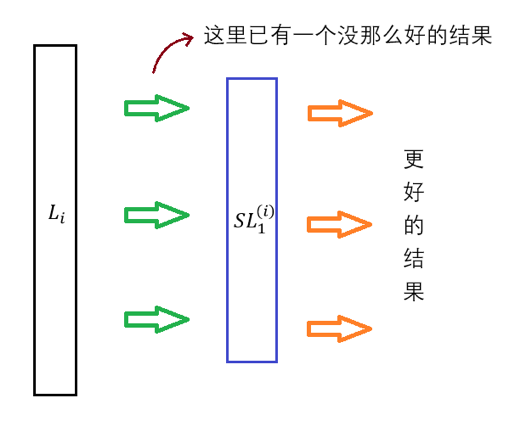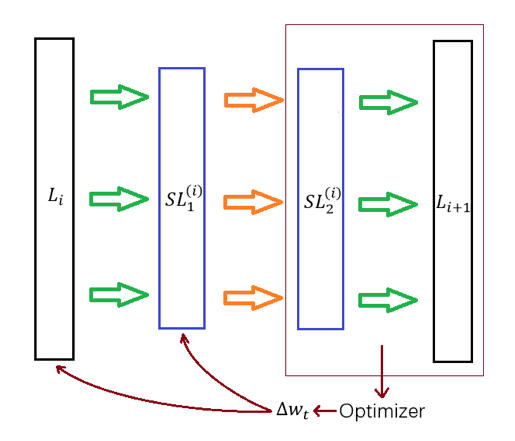典型的 SubLayer 有前文提到过的 Dropout 和 Normalize。它们都是近年来才提出的技术，其中 Dropout 是由 Srivastava 等人在 Journal of Machine Learning Research 15 (2014） 上的一篇论文中最先提出的、全文共 30 页，感兴趣的读者可以直接参见这里；Normalize 则是 Batch Normalization 对应的特殊层结构、它是由 Sergey loffe 和 Christian Szegedy 在 2015 年最先提出的，感兴趣的读者可以直接参见这里，这里仅直观地进行一些说明：

• Dropout 的核心思想在于提高模型的泛化能力：它会在每次迭代中依概率去掉对应 Layer 的某些神经元，从而每次迭代中训练的都是一个小的神经网络
• Normalize 的核心思想在于把父层的输出进行“归一化”、从而期望能够解决由于网络结构过深而引起的“梯度消失”等问题

虽说实现 SubLayer 本身并不是一个特别困难的任务，但是处理 SubLayer 之间的关联、SubLayer 与 Layer 之间的关联以及反向传播算法却是一件相当麻烦的事；具体的实现细节比较繁杂、这里就不进行叙述了。观众老爷们可以尝试按照上文相关的思想和定义来进行实现、我个人实现的版本则可以参见这里

注意：我们会在下个系列的文章中利用 Tensorflow 框架进行相关的实现，彼时我们会结合具体实现对 Dropout 和 Normalize 进行深入一些的介绍

至此、神经网络会用到的所有层结构就都大致说明了一遍，接下来就要解决一个至关重要但又还没解决的问题了：如何使用局部梯度来更新相应 Layer 中的参数

]]>
<p>在神经网络模型中有一类特殊的 Layer 结构——它们不会独立地存在、而会“依附”在某个 Layer 之后以实现某种特定的功能。一般我们会称这种特殊的 Layer 结构为附加层（SubLayer）</p>
反向传播算法 http://mlblog.carefree0910.me/posts/437097cd/ 2017-05-05T13:27:21.000Z 2017-05-06T02:24:18.000Z 本文要讲的就是可能最让我们头疼的反向传播（Backpropagation，常简称为 BP）算法了。事实上，如果不是要做理论研究而只是想快速应用神经网络来干活的话，了解如何使用 Tensorflow 等帮我们处理梯度的成熟的框架可能会比了解算法细节要更好一些（我们会把本章实现的模型的 Tensorflow 版本放在下一个系列中进行说明）。但即使如此，了解神经网络背后的原理总是有益的，在某种意义上它也能告诉我们应该选择怎样的神经网络结构来进行具体的训练

# 算法概述

顾名思义、BP 算法和前向传导算法的“方向”其实刚好相反：前向传导是由后往前（将激活值）一路传导，反向传播则是由前往后（将梯度）一路传播

注意：这里的“前”和“后”的定义是由 Layer 和输出层的相对位置给出的。具体而言，越靠近输出层的 Layer 我们称其越“前”、反之就称其越“后”

先从直观上理解一下 BP 算法的原理。总体上来说，BP 算法的目的是利用梯度来更新结构中的参数以使得损失函数最小化。这里面就涉及两个问题：

• 如何获得（局部）梯度？
• 如何使用梯度进行更新？

本节会简要介绍第一个问题应该如何解决、并说一种第二个问题的解决方案，对第二个问题的详细讨论会放在第 5 节中；正如前面提到的，BP 是在前向传导之后进行的、从前往后传播的算法，所以我们需要时刻记住这么一个要求——对于每个 Layer（$L_{i}$）而言、其（局部）梯度的计算除了能利用它自身的数据外、仅会利用到（假设包括输入、输出层在内一共有 m 个 Layer、符号约定与上述符号约定一致）：

• 上一层（$L_{i - 1}$）传过来的激活值$v^{\left( i - 1 \right)}$和下一层（$L_{i + 1}$）传回来的（局部）梯度$\delta^{\left( i + 1 \right)}$
• 该层与下一层之间的线性变换矩阵（亦即权值矩阵）$w^{\left( i \right)}$

其中出现的“局部梯度”的概念即为 BP 算法获得梯度的核心。其数学定义为：

一般而言我们会用其向量形式：

需要注意的是、此时数据样本数$N$不可忽视，亦即$u^{\left( i \right)}$$\delta^{\left( i \right)}$其实都是$N \times n_{i}$的矩阵。

由名字不难想象、局部梯度$\delta^{\left( i \right)}$仅在局部起作用且能在局部进行计算，事实上 BP 算法也正是通过将局部梯度进行传播来计算各个参数在全局的梯度、从而使参数的更新变得非常高效的。有关局部梯度的推导是相当繁复的工作、其中的细节我们会在相关数学理论中进行说明，这里就只叙述最终结果：

• BP 算法的第一步为得到损失函数的梯度： 注意式中运算符“”两边都是维的矩阵（其中即为输出层所含神经元的个数）、运算符“”本身代表的则是 element wise 操作，亦即若 则有 同理若
• BP 算法剩下的步骤即为局部梯度的反向传播过程： 这里列举出各个变量的维度以便理解：
• 局部梯度、激活函数的导数：$\delta^{\left( i \right)}$$\phi_{i}^{'}\left( u^{\left( i \right)} \right)$的维度为$N \times n_{i}$
• 权值矩阵的转置：$w^{\left( i \right)T}$的维度为$n_{i + 1} \times n_{i}$
• 局部梯度：$\delta^{\left( i + 1 \right)}$的维度为$N \times n_{i + 1}$

如果不管推导的话、求局部梯度的过程本身其实是相当清晰简洁的；如果所用的编程语言（比如 Python）能够直接支持矩阵操作的话、求解局部梯度的过程完全可以用一行实现

# 损失函数的选择

我们在上一篇文章中说过、损失函数通常需要结合输出层的激活函数来讨论，这是因为在 BP 算法的第一步所计算的局部梯度$\delta^{\left( m \right)}$正是由损失函数对模型输出$v^{\left( m \right)}$的梯度$\frac{\partial L\left( y,v^{\left( m \right)} \right)}{\partial v^{\left( m \right)}}$和激活函数的导数$\phi_{m}^{'}\left( u^{\left( m \right)} \right)$通过 element
wise 操作“*”得到的。不难想象对于固定的损失函数而言、会有相对“适合它”的激活函数，而事实上、结合激活函数来选择损失函数确实是一个常见的做法。用得比较多的组合有以下四个：

• Sigmoid 系以外的激活函数$+$距离损失函数（MSE）
MSE 可谓是一个万金油，它不会出太大问题、同时也基本不能很好地解决问题。这里特地指出不能使用 Sigmoid 系激活函数（目前我们提到过的 Sigmoid 系函数只有 Sigmoid 函数本身和 Tanh 函数），是因为 Sigmoid 系激活函数在图像两端都非常平缓（可以结合之前的图来理解）、从而会引起梯度消失的现象。MSE 这个损失函数无法处理这种梯度消失、所以一般来说不会用 Sigmoid 系激活函数$+$MSE 这个组合。具体而言，由于对 MSE 来说： 所以 结合 Sigmoid 的函数图像不难得知：若模型的输出$v^{\left( m \right)} \rightarrow \mathbf{0} = \left( 0,\ldots,0 \right)^{T}$但真值$y = \mathbf{1} = \left( 1,\ldots,1 \right)^{T}$；此时虽然预测值和真值之间的误差几乎达到了极大值、不过由于 从而 亦即第一步算的局部梯度就趋近于 0 向量了；可以想象在此场景下模型参数的更新将会非常困难、收敛速度因为会变得很慢。前文提到若干次的梯度消失、正是这种由于激活函数在接近饱和时变化过于缓慢所引发的现象
• Sigmoid$+$Cross Entropy
Sigmoid 激活函数之所以有梯度消失的现象是因为它的导函数形式为 想要解决梯度消失的话，比较自然的想法是定义一个损失函数、使得它导函数的分母上有$\phi\left( x \right)\left\lbrack 1 - \phi\left( x \right) \right\rbrack$这一项。而前文说过的 Cross Entropy 这个损失函数恰恰满足该条件、因为其导函数形式为 $v^{\left( m \right)} = \phi_{m}\left( u^{\left( m \right)} \right)$，从而有 这就相当完美地解决了梯度消失问题
• Softmax$+$Cross Entropy / log-likelihood
这两个组合的核心都在于前面额外用了一个 Softmax。Softmax 比起一个激活函数来说更像是一个（针对向量的）变换，它具有相当好的直观：能把模型的输出向量通过指数函数归一化成一个概率向量。比如若输出是$\left( 1,\ 1,\ 1,\ 1 \right)^{T}$，经过 Softmax 之后就是$\left( 0.25,\ 0.25,\ 0.25,\ 0.25 \right)^{T}$。它的严格定义式也比较简洁（以$\varphi$代指 Softmax）： 其中 从而 亦即 这和 Sigmoid 函数的导函数形式一模一样
之所以要进行这一步变换，其实是因为 Cross Entropy 用概率向量来定义损失（要比用随便一个各位都在内的向量）更好、且 log-likelihood 更是只能使用概率向量来定义损失。由于 Sigmoid$+$Cross Entropy 的求导已经介绍过且 Softmax 导函数与 Sigmoid 导函数一致、这里就只需给出 Softmax$+$log-likelihood 的求导公式： 亦即 其中 将该式写成向量化的形式并不容易、但从实现的角度来说却也不算困难（以上公式的推导过程会放在相关数学理论中）。不过需要注意的是，像这样算出来的局部梯度会是一个非常稀疏的矩阵（亦即大部分元素都是 0）、从而很容易导致训练根本无法收敛，这也正是为何前文说 log-likelihood 的原始形式不尽合理。改进的方法很简单、只需将损失函数变为： 即可。不难发现这个改进后的损失函数和 Cross Entropy 从本质上来说是一样的、所以我们在后文不会实现 log-likelihood 对应的算法

以上我们对如何获取局部梯度作了比较充分的介绍，对于如何利用局部梯度更新参数的详细讲解会放在第5节、这里仅介绍一种最简单的做法：直接应用上一章说过的随机梯度下降（SGD）。由于可以推出（推导过程同样可参见相关数学理论）：

从而只需

即可完成一步训练

# 相关实现

至此、神经网络中的 Layer 结构所需完成的所有工作就都已经介绍完毕，接下来就是归纳总结并着手实现的环节了。不难发现，每个 Layer 除了前向传导和反向传播算法核心以外，其余结构、功能等都完全一致；再加上这两大算法的核心只随激活函数的不同而不同、所以只需把激活函数留给具体的子类定义即可，其余的部分则都应该抽象成一个基类。由简入繁、我们可以先进行一个朴素的实现：

以上是对结构的抽象。由于我们实现的是一个比较朴素的版本、所以这个框架里也没有太多东西；如果要考虑上特殊的结构（比如后文会介绍的 Dropout、Normalize 等“附加层”）的话、就需要再往这个框架中添加若干属性

接下来就是对两大算法（前向传导、反向传播）的抽象（不妨设当前 Layer 为$L_{i}$）：

出于优化的考虑、我们在上述实现的bp方法中留了一些“余地”。具体而言，考虑到神经网络最后两层通常都是前文提到的 4 种组合之一、所以针对它们进行算法的优化是合理的；而为了具有针对性、CostLayer 的 BP 算法就无法包含在这个相对而言抽象程度比较高的方法里面。具体细节会在后文进行介绍、这里只说一下 CostLayer 自带的 BP 算法的大致思路：它会根据需要将相应的额外变换（比如 Softmax 变换）和损失函数整合在一起并算出一个整合后的梯度

以上便完成了 Layer 结构基类的定义，接下来就说明一下为何在定义derivative这个计算激活函数导函数的方法时、传进去的参数是该 Layer 的输出值$v^{\left( i \right)} = \phi_{i}\left( u^{\left( i \right)} \right)$。其实理由相当平凡：很多常用的激活函数的导函数使用函数值来定义会比使用自变量来定义要更好（所谓更好是指形式上更简单、从而计算开销会更小）。接下来就罗列一下上文提到过的、6 种激活函数的导函数的形式：

• 逻辑函数（Sigmoid）
• 正切函数（Tanh）
• 线性整流函数（Rectified Linear Unit，常简称为 ReLU）
• ELU 函数（Exponential Linear Unit）
• Softplus 函数
• 恒同映射（Identity）

可以看出，用$\phi(x)$来表示$\phi'(x)$确实基本都比用$x$来表示$\phi'(x)$要简单、高效不少，所以在传参时将激活函数值传给计算导函数值的方法是合理的

接下来就是实现具体要用在神经网络中的 Layer 了；由前文讨论可知、它们只需定义相应的激活函数及（用激活函数值表示的）导函数即可。以经典的 Sigmoid 激活函数所对应的 Layer 为例：

其余 5 个激活函数对应 Layer 的实现是类似的、观众老爷们可以尝试对照着公式进行实现，我个人实现的版本则可以参见这里

最后我们要实现的就是那有些特殊的 CostLayer 了。总结一下前文所说的诸多内容、可知实现 CostLayer 时需要注意如下两点：

• 没有激活函数、但可能会有特殊的变换函数（比如说 Softmax），同时还需要定义某个损失函数
• 定义导函数时，需要考虑到自身特殊的变换函数并计算相应的、整合后的梯度

具体的代码也是非常直观的，先来看看其基本架构：

接下来就要定义相应的变换函数了。由前文对四种损失函数组合的讨论及上述代码都可以看出、我们需要定义 Softmax 和 Sigmoid 这两种变换函数及相应导函数：

其中前三行代码实现的safe_exp方法主要利用了如下恒等式：

其中$c$是任意一个常数；如果此时我们取

这样的话分母、分子中所有幂次都不大于 0，从而不会出现由于某个$v_{i}^{\left( m \right)}$很大而导致对应的$e^{v_{i}^{\left( m \right)}}$很大、并因而导致数据溢出的情况，从而在一定程度上保证了数值稳定性

接下来要实现的就是各种损失函数以及能够根据损失函数计算整合梯度的方法了；考虑到可拓展性，我们不仅要优化特定的组合对应的整合算法、同时也要考虑一般性的情况。因此在实现损失函数的同时、实现损失函数的导函数是有必要的：

至此、我们打算实现的朴素神经网络模型中的所有 Layer 结构就都实现完毕了。下一节我们会介绍一些特殊的 Layer 结构，它们不会整合在我们的朴素神经网络结构中；但是如果想在实际任务中应用神经网络的话、了解它们是有必要的

]]>
<p>本文要讲的就是可能最让我们头疼的反向传播（Backpropagation，常简称为 BP）算法了。事实上，如果不是要做理论研究而只是想快速应用神经网络来干活的话，了解如何使用 Tensorflow 等帮我们处理梯度的成熟的框架可能会比了解算法细节要更好一些（我们会把本章实现的模型的 Tensorflow 版本放在下一个系列中进行说明）。但即使如此，了解神经网络背后的原理总是有益的，在某种意义上它也能告诉我们应该选择怎样的神经网络结构来进行具体的训练</p>
前向传导算法 http://mlblog.carefree0910.me/posts/2a8cdd6/ 2017-05-05T12:51:11.000Z 2017-05-06T02:15:04.000Z 时至今日，在各个编程语言的世界里、神经网络的成熟的库都可谓不在少数；这可能就导致有许多人虽然能够熟练应用神经网络、但对于其内部机制却不甚了解。事实上就笔者所展开的简单调查来看，有不少平时经常用到神经网络的程序员其实对神经网络的数学部分有一种“望而生畏”的感觉、其中各种梯度的计算更是让他们发出“眼花缭乱”的感叹

虽然很想说一些令人鼓舞的话，但是如果从繁复性来说、神经网络算法确实是我们目前为止介绍过的算法中推导步骤最多的；不过可以保证的是，如果把算法的逻辑理清，那么静下心来好好演算一下的话、就会觉得它比想象中的简单

# 算法概述

如果把前文所说过的内容提炼、总结一下的话，就会发现我们其实已经把前向传导算法的过程都叙述了一遍。以一个简单的神经网络结构为例：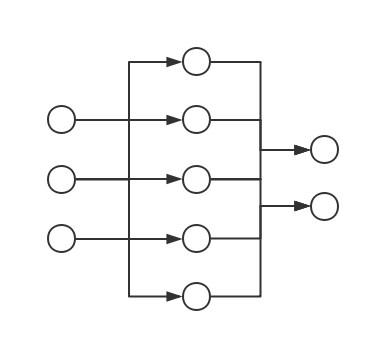注意：虽然上图将一个个的“节点”画了出来，但是本篇文章及今后的所有讨论中、我们都应该时刻记住：神经网络的基本组成单元是层（Layer）而不是节点，之所以用节点来说明问题也仅仅是为了简化问题、在实现中是需要将节点上的算法“整合”成层的算法的

在展开叙述前、做一些符号约定是有必要的：

• 记上图中的神经网络从左到右对应的 Layer 为$L_{1},L_{2},L_{3}$、记$L_{i}$中从上往下数的第 j 个神经元为$u_{ij}$
• $L_{i}$对应的：
• 神经元个数为$n_{i}$（从而$n_{1} = 3$$n_{2} = 5$$n_{3} = 2$
• 激活函数、偏置量分别为$\phi_{i}$$b^{\left( i \right)}$（注意$b^{\left( 3 \right)}$其实不会被用到）
• $L_i,L_{i+1}$之间的权值矩阵为$w^{(i)}$、神经元$u_{ij},u_{i+1,k}$之间的权值为$w_{jk}^{(i)}$，可知：
• $L_{i}$对应的输入、输出为$u^{\left( i \right)},v^{\left( i \right)}$
• 记模型的输入、输出集为$X$$Y$，样本数为 N，损失函数为$L$；一般我们会要求$L$是一个二元对称函数，亦即对于$L$的输入空间中的任意两个向量（矩阵）$p$$q$都有：

那么上述神经网络的前向传导算法的所有步骤即为（运算符“$\times$”代表矩阵乘法、后同）：

• $u^{\left( 1 \right)} = X$$v^{\left( 1 \right)} = \phi_{1}(u^{\left( 1 \right)})$，注意$u^{\left( 1 \right)}$$v^{\left( 1 \right)}$都是$N \times 3$维矩阵
• $u^{\left( 2 \right)} = v^{\left( 1 \right)} \times w^{\left( 1 \right)} + b^{\left( 1 \right)}$$v^{\left( 2 \right)} = \phi_{2}(u^{\left( 2 \right)})$，注意$w^{\left( 1 \right)}$$3 \times 5$的矩阵、所以$u^{\left( 2 \right)}$$v^{\left( 2 \right)}$都是$N \times 5$维矩阵
• $u^{\left( 3 \right)} = v^{\left( 2 \right)} \times w^{\left( 2 \right)} + b^{\left( 2 \right)}$$v^{\left( 3 \right)} = \phi_{3}(u^{\left( 3 \right)})$，注意$w^{\left( 2 \right)}$$5 \times 2$的矩阵、所以$u^{\left( 3 \right)}$$v^{\left( 3 \right)}$都是$N \times 2$维矩阵

其中$v^{\left( 3 \right)}$即为模型的输出、$L\left( v^{\left( 3 \right)},Y \right) = L\left( Y,v^{\left( 3 \right)} \right)$即为模型在$(X,Y)$上的损失。可以看到这个过程确实相当平凡、但是里面蕴含的数学思想却是有趣而深刻的，接下来我们就分析一下其中的一些细节

注意：以上这个例子中的神经网络模型其实是一个二分类模型（$n_{3} = 2$），如果想用神经网络解决多分类问题（比如 K 分类问题）的话、只需自然地将输出层的神经元个数设为类别个数（$n_{3} \leftarrow K$）即可。此外，简便起见，如果我们没有特别指出的话、那么下文中所讨论的情况都是$N = 1$、亦即样本集里只有单样本的情形

# 激活函数

首先说说前文不断在提却又没有细说的激活函数$\phi$。直观来讲，所谓激活函数、正是整个结构中非线性扭曲力。这里介绍几个常见的激活函数：

## 逻辑函数（Sigmoid）

其函数图像如下图所示：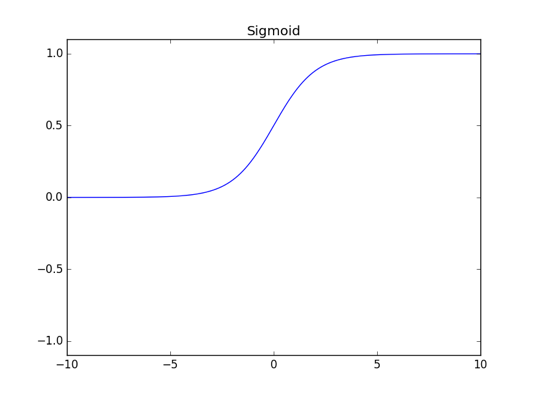## 正切函数（Tanh）

其函数图像如下图所示：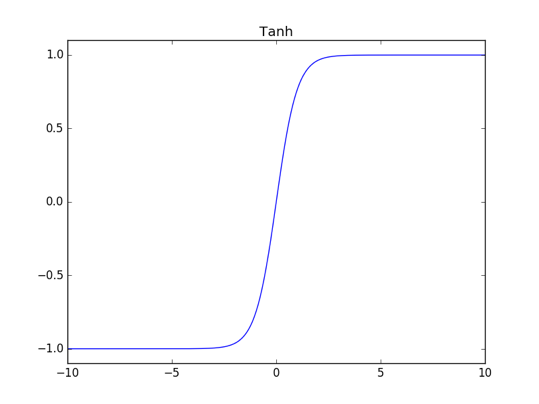## 线性整流函数（ReLU）

ReLU 的全称是 Rectified Linear Unit，定义式很简洁：

其函数图像如下图所示（注意纵轴范围与上述两个激活函数不同）：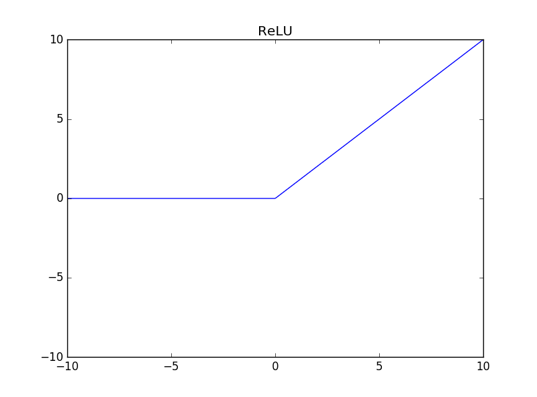## ELU 函数（Exponential Linear Unit）

我们在实现时会取$\alpha=1$、其函数图像如下图所示：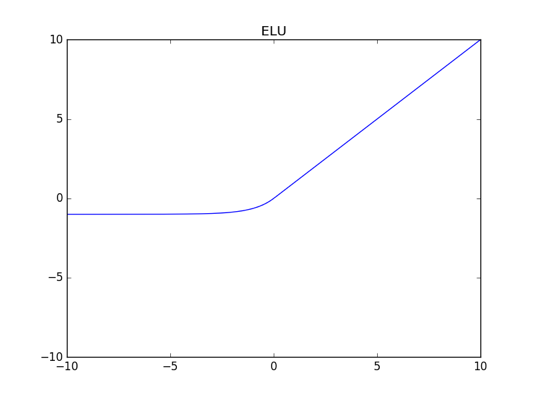## Softplus

其函数图像如下图所示：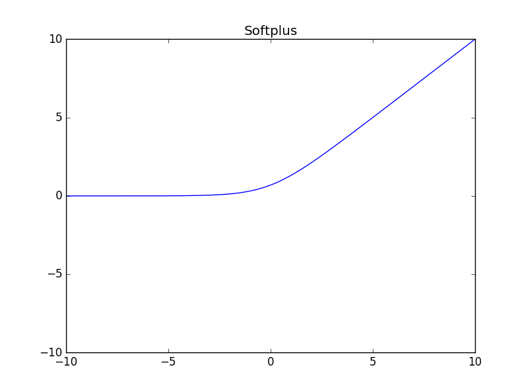## 恒同映射（Identify）

其函数图像从略

囿于篇幅、这些激活函数的由来及背后相关的错综复杂的数学理论研究就不展开叙述了；我们只需知道，神经网络之所以为非线性模型的关键、其实就在于激活函数

然后来看看层与层之间的权值矩阵$w$以及偏置量$b$、它们的意义也都有比较好的解释：

• $w$能把从激活函数得到的函数值线性映射到另一个维度的空间上
• $b$能在此基础上再进行一步平移的操作

其中$w$的重要性似乎无需过多说明也能让人明白，但$b$的重要性相对而言可能就没那么明显。为了直观体会偏置量$b$的重要性、可以设想这么一个场景（取之前的三层网络结构来说明问题）：

• 激活函数全是中心对称的函数（比如常见的 tanh 函数）、亦即：
• 训练样本集为：
• 权值矩阵$w^{\left( 1 \right)}$$w^{\left( 2 \right)}$可变但偏置量恒为 0

在此场景下不难想象，无论我们怎样进行训练、模型$G$在训练集$D$上的准确率都不可能达到 100%。这是因为我们有：

从而由激活函数为中心对称函数可知：

亦即

但我们有$y_{1} = y_{2} = - 1$、所以模型$G$不可能同时预测对$(x_{1},y_{1})$$(x_{2},y_{2})$。事实上由上述讨论可知、此时模型$G$所做的预测必定是关于输入空间“中心对称”的，这当然不是一个良好的结果。而如果我们引入偏置量的话、上述的对称性就会被打破，这就是偏置量重要性的其中一个比较浅显、直观的方面

# 损失函数（Cost Function）

注意到前向传导算法的最后一步是将模型的输出与真值相比较、并通过损失函数的作用来得到一个损失。损失函数有时也写作 Loss Function、我们之前已经提及它许多次。损失函数的直观意义是明确的：它是模型对数据拟合程度的反映；拟合得越差、损失函数的值就应该越大。如果同时考虑到梯度下降法的应用、我们自然还应该期望，当损失函数在函数值比较大（亦即模型的表现越差）时、它对应的梯度也要比较大（亦即更新参数的幅度也要比较大）

由于我们此前没有对梯度下降法进行过深刻的应用（上个系列中的随机梯度下降只是一个相当粗浅的应用）、所以至今为止我们涉及到的损失函数基本只满足了“模型越差函数值越大”这一点，对于“函数值越大则梯度越大”这一点则没怎么考虑到。而对于神经网络而言、梯度下降可谓就是训练的全部，时至今日也没能出现能够与之抗衡的其余算法、最多也只是不断地研究出各式各样的梯度下降法的变体而已；所以对于神经网络来说，定义一个足够合适的损失函数是有必要的。接下来就介绍其中最常用的几个，为此需要先做符号约定：

• 假设样本为$(x,y)$
• 假设共有 K 类：$\{ c_{1},\ldots,c_{K}\}$
• 假设讨论的模型为$G$、其输出（向量）为$G(x)$

其中$x \in \mathbb{R}^{n}$$y \in \mathbb{R}^{K}$，且$y$是除了一位为 1、其余位都是 0 的向量。换句话说，若$y \in c_{k}$、那么$y$除了第 k 位为 1、其余位都是 0

注意：这种$y$的表示方法通常叫做 one-hot representation

在神经网络的训练算法中、损失函数通常需要结合输出层的激活函数来讨论；不过如果只考虑前向传导算法、只叙述损失函数的基本形式就可以：

• 距离损失函数 该损失函数对应着最小平方误差准则（Minimum Squared Error，常简称为 MSE），它的直观意义是明确的：模型预测和真值的（欧氏）距离越大、损失就越大，反之就越小
• 交叉熵损失函数（要求$G(x)$每一位的取值都在$(0,1)$中） 其中交叉熵（Cross Entropy）是信息论中的一个概念、其本身是有一定内涵的，感兴趣的观众老爷可以参见维基百科来了解背后的那一套数学理论。囿于篇幅、我们无法展开叙述这一部分，但是从交叉熵的名字就可以看出、它和决策树里面提到过的熵有千丝万缕的关系；考虑到熵是定义在概率分布上的、所以进一步要求是一个概率向量（亦即进一步要求$\sum_{k=1}^Kv_k=1$）是一个非常合理的做法
• log-likelihood 损失函数（要求$G(x)$是一个概率向量、以及不妨假设$y\in c_k$换句话说，log-likelihood 即为模型预测的、真值 y 对应的类（$c_k$）的概率的负对数。需要指出的是、log-likelihood 这种原始的定义方式在神经网络里面不尽合理，我们会在下一节进行相关的讨论

以上、我们就比较完整地叙述了一遍前向传导算法。可以看出在前向传导算法中、神经网络的各个 Layer 结构在很多地方的表现都一致、所以把 Layer 的共性抽象出来是有必要的。事实上再通过后面对神经网络的训练算法（反向传播算法）的说明我们就可以看出，每个变换层除了所对应的激活函数有所不同以外、其余部分的表现都几乎一样；而 CostLayer 虽然表现会有点不同（比如需要额外考虑损失函数、从而导致反向传播的形式会有些许改变）、其总体结构仍与变换层大致相同

]]>
<p>时至今日，在各个编程语言的世界里、神经网络的成熟的库都可谓不在少数；这可能就导致有许多人虽然能够熟练应用神经网络、但对于其内部机制却不甚了解。事实上就笔者所展开的简单调查来看，有不少平时经常用到神经网络的程序员其实对神经网络的数学部分有一种“望而生畏”的感觉、其中各种梯度的计算更是让他们发出“眼花缭乱”的感叹</p> <p>虽然很想说一些令人鼓舞的话，但是如果从繁复性来说、神经网络算法确实是我们目前为止介绍过的算法中推导步骤最多的；不过可以保证的是，如果把算法的逻辑理清，那么静下心来好好演算一下的话、就会觉得它比想象中的简单</p>
从感知机到多层感知机 http://mlblog.carefree0910.me/posts/3fa9a563/ 2017-05-05T12:25:50.000Z 2017-05-19T16:47:10.000Z “神经网络”这个概念本身其实是一个庞大的交叉学科，而在机器学习领域里、“神经网络”则是“人工神经网络（Artificial Neural Network）”的简称。顾名思义，本系列所将介绍的神经网络模型多少借鉴了神经生理学关于神经网络的研究、并尝试通过数学建模来描述机器智能，这也正是为何许多机器学习相关书籍对神经网络的介绍都会从“真正的”神经网络开始（比如介绍细胞体、树突、轴突和突触之类的）。然而个人认为，直到目前为止、我们一般应用的神经网络结构其实和真正的神经网络之结构之间的差距还是相当大的；如果按照生物学意义上的神经网络来理解人工神经网络的话，虽说从直观上来说可能更加易懂、但在逻辑和原理的层面上反而会造成混淆

为此我们就跳过“老生常谈”般的、介绍生物学意义上的神经网络的部分并直接把数学建模后的结果进行说明：近现代最常用的NN模型其实脱胎于 1943 年由 W. S. McCulloch 和 W. H. Pitts 提出的 McCulloch-Pitts 神经元模型（常简称为 M-P 神经元模型），它针对单个的神经元进行了数学建模。具体而言、M-P 模型是具有如下三个功能的模型：

• 能够接收 n 个 M-P 模型传递过来的信号
• 能够在信号的传递过程中为信号分配权重
• 能够将得到的信号进行汇总、变换并输出

可以通过下图来直观认知 M-P 模型的结构：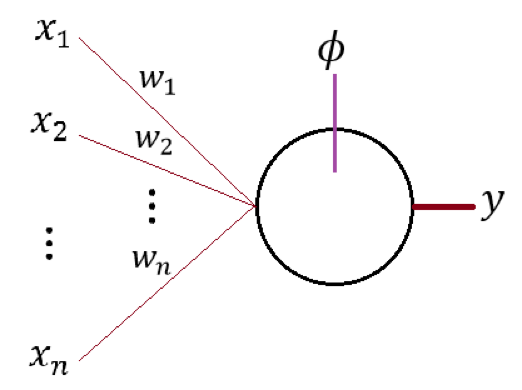图中的$x_{1},\ldots,x_{n}$即为 n 个 M-P 模型的输出信号、$w_{1},\ldots,w_{n}$即为这 n 个信号对应的权值；$\phi$即为所示神经元对输入信号的变换函数、y 即为模型的输出。一般而言我们可以把 y 写成：

其中 b 为神经元对输入信号的“平移”。我们通常会称$\phi$为激活函数而称 b 为偏置量，有关它们的详细讨论会在前向传导算法中进行、这里就暂时先按下不表

有了 M-P 神经元模型的话、基于它来定义神经网络似乎就不是一件困难的事了；事实上、只需要把许多 M-P 神经元按照一定的层次结构进行连接即可。一个非常自然的想法就是构建一个有向无环图（DAG 图），其输入节点和输出节点视具体问题而定。比如若想通过三维的输入来得到二维的输出、我们可以简单地以 M-P 模型为有向无环图中的节点来构造一个如下图所示的有向无环图：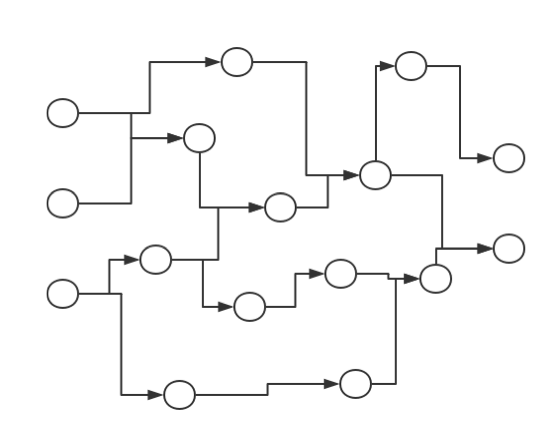如果人工神经网络模型真的能够对任意 DAG 图都能进行高效训练的话、那么说它和真正的神经网络能够互相类比可能也不算夸张；然而遗憾的是，由于现在我们对矩阵运算的依赖程度很大（因为矩阵运算是被高度优化了的），所以目前主流的神经网络模型结构基本都是一类及其特殊的 DAG 图。具体而言、主流人工神经网络模型是以“层（Layer）”（而不是以“节点”）为基本单位的，其结构大致如下图所示：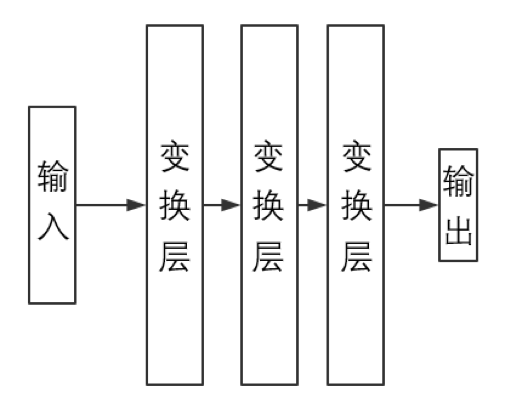其中，输入（层）、变换层和输出（层）都可以想象为是若干 M-P 神经元“排列在一起”而组成的“神经层”、从而整张神经网络即为由若干神经层“堆叠而成”的一个结构。不难想象在这种情况下、同一层中的所有 M-P 神经元会共享激活函数$\phi$和偏置量 b，所以通常我们会针对层结构定义$\phi$和 b 而不是针对单个的神经元定义$\phi$和 b

如果确实想以“节点”为基本单位、那么上图所示结构可以化为如下图所示的模型：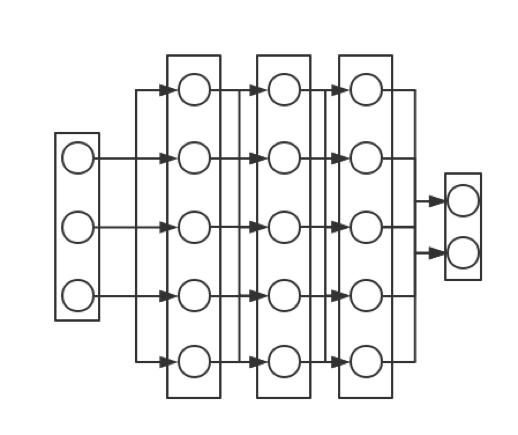其中除了输出层外、当前层的每个节点都会出来一个箭头指向下一层中的每个节点，这也正是当前层将信号传输给下一层的方式。容易想象当没有变换层时、人工神经网络就会“退化”成我们上个系列中讲过的感知机。事实上可以将第一张图所示的神经元看作是只有一个神经元的输出层并令$\phi$为恒同映射、亦即：

那么就有

其中

可以看出上式即为感知机的决策公式。由此可见、这种主流人工神经网络结构其实可以称为多层感知机模型（Multi-Layer Perceptron，常简称为 MLP），本章所说的神经网络所代指的也正是 MLP 模型。它的工作原理是直观的：

• 输入层和输出层即为整个模型的入口和出口
• 变换层则会把上一层的输出当成输入、经过一番内部处理后把输出传给下一层

所以问题的关键就在于层结构（Layer）的搭建上。不过在着手实现它之前、了解它具体需要做哪些工作是有必要的。如果往简单去说、神经网络算法其实只包含如下三个部分：

• 通过将输入进行一层一层的变换来得到输出
• 通过输出与真值的比较得到损失函数的梯度
• 利用得到的这个梯度来更新模型的各个参数

其中前两个部分相关的内容会在下两节进行简单的说明、第三个部分相关内容的简要叙述则会放在第四节。注意到第二个部分中提到了“损失函数”的概念；在我们将要实现的神经网络模型中、我们会将损失作为一个单独的层结构跟在输出层后面。换句话说、一个完整的神经网络模型将如下图所示：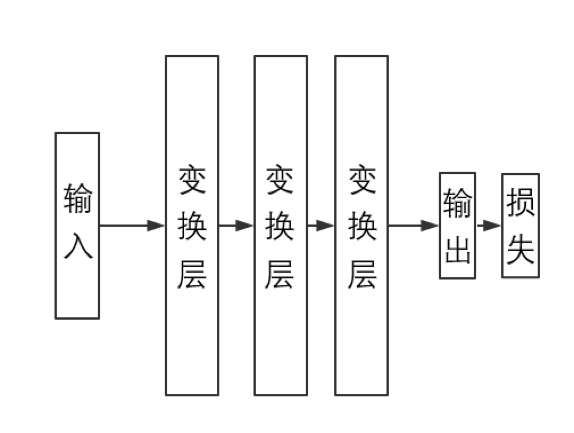注意：今后章节中出现的各个数学算式中的元素如果不带下标的话、一般而言都代指向量或者矩阵而不是标量；为使文章结构连贯，我们不会一一说明哪些是标量、哪些是向量而哪些是矩阵，但是通过上下文和具体的算法、相关叙述应该是不会引起歧义的

此外需要指出的是，由于损失层 CostLayer 只是为了实现的便利性而存在的结构、从数学的角度来讲它是不必抽出来作为一个独立个体的。因此我们有时会在叙述数学相关问题时会隐去 CostLayer

]]>
<p>“神经网络”这个概念本身其实是一个庞大的交叉学科，而在机器学习领域里、“神经网络”则是“人工神经网络（Artificial Neural Network）”的简称。顾名思义，本系列所将介绍的神经网络模型多少借鉴了神经生理学关于神经网络的研究、并尝试通过数学建模来描述机器智能，这也正是为何许多机器学习相关书籍对神经网络的介绍都会从“真正的”神经网络开始（比如介绍细胞体、树突、轴突和突触之类的）。然而个人认为，直到目前为止、我们一般应用的神经网络结构其实和真正的神经网络之结构之间的差距还是相当大的；如果按照生物学意义上的神经网络来理解人工神经网络的话，虽说从直观上来说可能更加易懂、但在逻辑和原理的层面上反而会造成混淆</p>
神经网络综述 http://mlblog.carefree0910.me/posts/d94622d/ 2017-05-05T02:20:11.000Z 2017-05-06T01:52:41.000Z 之前所介绍的算法可算是比较“经典”、“传统”的算法；它们其实都属于统计学习方法、有着相当深厚的统计学理论作为支撑。而本章所讲的神经网络（Neural Network，常简称为 NN）则是近代比较火热的算法。尽管该算法的提出已经颇有些年头，相应的数学理论亦提出了不少，而且也有不少人认为它归于统计学习方法，但相当多的人还是认为，该算法更像一门“手艺”

以下是目录：

]]>
<p>之前所介绍的算法可算是比较“经典”、“传统”的算法；它们其实都属于统计学习方法、有着相当深厚的统计学理论作为支撑。而本章所讲的神经网络（Neural Network，常简称为 NN）则是近代比较火热的算法。尽管该算法的提出已经颇有些年头，相应的数学理论亦提出了不少，而且也有
“支持向量机”小结 http://mlblog.carefree0910.me/posts/5b3e9c59/ 2017-04-28T11:25:48.000Z 2017-04-28T11:26:50.000Z
• 感知机利用 SGD 能保证对线性可分数据集正确分类（无论学习速率为多少）、但它没怎么考虑泛化能力的问题
• 线性 SVM 通过引入间隔（硬、软）最大化的概念来增强模型的泛化能力
• 核技巧能够将线性算法“升级”为非线性算法，通过将原始问题转化为对偶问题能够非常自然地对核技巧进行应用
• 对于一个二分类模型，有许多方法能够直接将它拓展为多分类问题
• SVM 的思想能用于做回归（SVR）；具体而言、SVR 容许模型输出和真值之间存在$\epsilon$的差距以期望提高泛化能力
• ]]>
<ul> <li>感知机利用 SGD 能保证对线性可分数据集正确分类（无论学习速率为多少）、但它没怎么考虑泛化能力的问题</li> <li>线性 SVM 通过引入间隔（硬、软）最大化的概念来增强模型的泛化能力</li> <li>核技巧能够将线性算法“升级”为非线性算法，通过将原始
相关数学理论 http://mlblog.carefree0910.me/posts/18dd8b30/ 2017-04-28T11:01:43.000Z 2017-07-03T15:42:21.000Z 这篇文章会叙述之前没有解决的纯数学问题，会涉及到相当庞杂的数学概念与思想，其中一些推导的难度相对而言可能会比较大

# 梯度下降法

前文已经相当充分地说明了梯度下降的直观，本节则打算用较严谨的数学语言来重新叙述一遍这个方法

首先说明其地位：梯度下降法（又称最速下降法）是求解无约束最优化问题的最常用的手段之一，同时由于现有的深度学习框架（比如 Tensorflow）基本都会含有自动求导并更新参数的功能、所以梯度下降法的实现往往会简单且高效

其次说明一下梯度下降法的大致步骤。正如前文所说、梯度下降法的核心在于在于函数的“求导”，而由于一般来说样本都是高维的样本（亦即$x \in \mathbb{R}^{n}$$n \geq 2$）、所以此时我们要求的其实是函数的梯度。由于梯度是微积分里面的基础知识、这里就不“追本溯源”般地讲解梯度的定义之类的了，如果确实不甚了解且不满足于前文给出的直观解释的话、可以参见维基百科中的详细定义（中文版英文版都有，个人建议尽量看英文版）

不管怎么说、函数梯度的这一点性质需要谨记：它是使函数值上升最快的方向，这就同时意味着负梯度是使函数值下降最快的“更新方向”。利用该性质，梯度下降法认为在每一步迭代中、都应该以梯度为更新方向“迈进”一步；在机器学习中、我们通常把这时迈进的“步长”称作“学习速率”：

1. 输入：想要最小化的目标函数$f(x)$、迭代次数 M、学习速率$\eta$、计算精度$\epsilon$，其中$x \in \mathbb{R}^{n}$
2. 过程
1. 求出$f(x)$的梯度函数：
2. 取一个初始估计值$x^{\left( 0 \right)} \in \mathbb{R}^{n}$
3. $j = 1,\ldots,M$
1. 计算负梯度——$g_{j} = - g(x^{\left( j \right)})$，若$\left\| g_{j} \right\| < \epsilon$则退出循环、令最终解$x^{*} = x^{\left( j \right)}$
2. 否则、向更新方向$g_{j}$迈进步长为$\eta$的一步：
3. $\left\| f\left( x^{\left( j + 1 \right)} \right) - f(x^{\left( j \right)}) \right\| < \epsilon$$\left\| x^{\left( j + 1 \right)} - x^{\left( j \right)} \right\| < \epsilon$则退出循环、令最终解$x^{*} = x^{\left( j + 1 \right)}$
3. 输出：最终解$x^{*}$

上述算法是一个最为朴素的梯度下降法框架，通过在其基础上结合具体的模型进行改进、拓展能够衍生出一系列著名的算法。具体而言、这些拓展算法通常会针对如下两个部分进行改进：

• 不是单纯地把梯度作为更新方向、而是利用更多的属性来定出更新方向
• 不把学习速率设成常量、而设法让其能够“适应算法”并根据具体情况进行调整

有关梯度下降的拓展算法会在下一个系列的文章中进行比较详细的叙述，这里我们仅针对第二点来举一个非常直观的改进例子（仅写出与上述算法中不同的部分）：

• 算法 2.3.2 步
$j = 1,\ldots,M$
• 否则求出$\eta_{j}$、使得： 然后根据$\eta_{j}$来更新估计值： 这种算法又可以称作精确线性搜索准则。当优化问题为凸优化、亦即函数为凸函数时，可以证明若迭代次数 M 足够大、精确线性搜索必定能够收敛到全局最优解

考虑到对于具体的机器学习模型而言、其训练时一般会同时用到许多的样本，此时进行梯度下降法的话就不免会遇到一个问题：计算梯度时，是应该同时对多个样本进行求解然后将结果整合、还是对样本逐个进行求解？对该问题的不同解答对应着不同的算法、前文也已经有所提及。具体而言：

• 对于随机梯度下降（SGD）、其求梯度的公式为： 其中 i 是一个合适的下标。SGD 的优缺点都比较直观：虽然（在同样的迭代次数下）它的训练速度很快、但它搜索解空间的过程会显得比较盲目（就有种东走一下西走一下的感觉），这直接导致其收敛速度反而可能会更慢。同时如果考虑实际应用的话，由于 SGD 难以并行实现、所以其效率往往会比较低
• 对于小批量梯度下降（MBGD）、其求梯度的公式为： 其中 m 是一个合适的、小于总样本数 N 的数，$S_1,...,S_m$则是 m 个合适的下标；通常我们会称$\left\{ x_{S_1},...,x_{S_m}\right\}$为一个 batch。MBGD 可谓是应用得最广泛的梯度下降法，它在单步迭代中会比 SGD 慢、但它对解空间的搜索会显得“可控”很多、从而收敛速度一般反而会比 SGD 要快
• 对于批量梯度下降（BGD）、其求梯度的公式为： BGD 会有一种“过犹不及”的感觉，由于它单步迭代中会用到所有样本，所以当训练集很大的时候、无论是时间开销还是空间开销都会变得难以忍受

以上我们就大概综述了一遍梯度下降法的框架，更为细致的具体算法则会在下一个系列中介绍神经网络时进行部分说明

# 拉格朗日对偶性

如果按照最一般性的定义来讲的话，拉格朗日对偶性会显得太过“纯粹”、或说可以算是数学家的游戏。因此本小节拟打算通过推导如何将软间隔最大化 SVM 的原始最优化问题转化为对偶问题、来间接说明拉格朗日对偶性的一般性步骤

注意到原始问题为：

使得：

其中

那么原始问题的拉格朗日函数即为：

为求解$L$的极小、我们需要对$w$$b$$\xi$求偏导并令偏导为 0。易知：

解得

以及对$i = 1,\ldots,N$、都有

将它们带入$L\left( w,b,\xi,\alpha,\beta \right)$、得

从而原始问题的对偶问题即为求上式的极大值、亦即

其中约束条件为：

以及对$i = 1,\ldots,N$、都有

易知上述约束可以简化为对$i = 1,\ldots,N$、都有

综上所述即得前文叙述过的软间隔最大化的对偶形式。注意到原始问题是凸二次规划、从而对偶形式的解$w^{*}$$b^{*}$$\xi^{*}$$\alpha^{*}$$\beta^{*}$满足 KKT 条件，亦即：

以及对$i = 1,\ldots,N$、都有

由它们就可以推出前文说明过的、$w^{*}$$b^{*}$关于$\alpha^{*}$的表达式了

]]>
<p>这篇文章会叙述之前没有解决的纯数学问题，会涉及到相当庞杂的数学概念与思想，其中一些推导的难度相对而言可能会比较大</p>
多分类与支持向量回归 http://mlblog.carefree0910.me/posts/1dc4445a/ 2017-04-28T10:43:38.000Z 2017-04-28T12:19:38.000Z 本篇文章将简要说明几种将二分类模型拓展为多分类模型的普适性方法，它们不仅能对前三篇文章叙述的感知机和 SVM 进行应用、同时还能应用于在上一个系列中进行了说明的 AdaBoost 二分类模型；在本篇的第四节（也是最后一节）、我们则会简要地说明一下如何将支持向量机的思想应用在回归问题上

# 一对多方法（One-vs-Rest）

一对多方法常简称为 OvR、是一种比较比较“豪放”的方法：对于一个 K 类问题、OvR 将训练 K 个二分类模型$\{ G_{1},\ldots,G_{K}\}$，每个模型将训练集中的某一类的样本作为正样本、其余类的样本作为负样本。模型的输出空间为实数空间、它反映了模型对决策的“信心”

具体而言、模型$G_{i}$会把第$i$类看成一类、把其余类看成另一类并尝试通过训练来区分开第$i$类和剩余类别；若$G_{i}$有比较大的自信来判定输入样本x是（或不是）第$i$类、那么$G_{i}(x)$将会是一个比较大的正（负）数，否则、$G_{i}(x)$将会是一个比较小的正（负）数

训练好 K 个模型后、直接将输出最大的模型所对应的类别作为决策即可、亦即：

之所以称这种方法比较“豪放”、主要是因为对每个模型的训练都存在比较严重的偏差：正样本集和负样本集的样本数之比在原始训练集均匀的情况下将会是$\frac{1}{K - 1}$。针对该缺陷、一种比较常见的做法是只抽取负样本集中的一部分来进行训练（比如抽取其中的三分之一）

# 一对一方法（One-vs-One）

一对一方法常简称为 OvO、可谓是一种很直观的方法：对于一个 K 类问题、OvO 将直接训练出$\frac{K\left( K - 1 \right)}{2}$个二分类模型$\{ G_{12},\ldots,G_{1K},G_{23},\ldots,G_{2K},\ldots,G_{K - 1,K}\}$，每个模型都只从训练集中接受两个类的样本来进行训练。模型的输出空间为二值空间$\{ - 1, + 1\}$、亦即模型只需要具有投票的能力即可

具体而言、模型$G_{\text{ij}}(i < j)$将接受且仅接受所有第$i$类和第$j$类的样本并尝试通过训练来区分开第$i$类和第$j$类；同时，假设$c_{i}$代表第$i$类的样本空间、那么就有：

训练好$\frac{K\left( K - 1 \right)}{2}$个模型后，OvO 将通过投票表决来进行决策、在$\frac{K\left( K - 1 \right)}{2}$次投票中得票最多的类即为模型所预测的结果。具体而言，如果考察$G_{ij}$、那么若$G_{\text{ij}}$输出$- 1$则第$j$类得一票、若$G_{ij}$输出$+ 1$则第$i$类得一票。如果只有两个类别（比如第$i$类和第$j$类）得票一致、那么直接看针对这两个类别的模型（亦即$G_{ij}$）的结果即可；如果多于两个类别的得票一致、则需要具体情况具体分析

OvO 是一个相当不错的方法、没有类似于 OvR 中“有偏”的问题。然而它也是有一个显而易见的缺点的——由于模型的量级是$K^{2}$、所以它的时间开销会相当大

# 有向无环图方法（Directed Acyclic Graph Method）

有向无环图方法常简称为 DAG，它的训练过程和 OvO 的训练过程完全一致、区别只在于最后的决策过程。具体而言、DAG 会将$\frac{K\left( K - 1 \right)}{2}$个模型作为一个有向无环图中的$\frac{K\left( K - 1 \right)}{2}$节点并逐步进行决策。其工作原理可以用下图进行说明（假设$K = 4$）：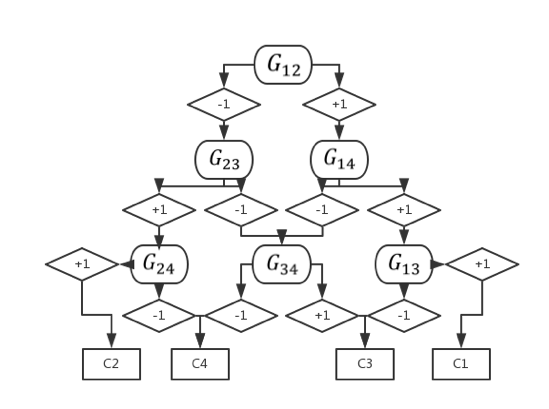# 支持向量回归（Support Vector Regression）

支持向量回归常简称为 SVR，它的基本思想与“软”间隔的思想类似——传统的回归模型通常只有在模型预测值$f(x)$和真值$y$完全一致时损失函数的值才为 0（最经典的就是当损失函数为$\left\| f\left( x \right) - y \right\|^{2}$的情形），而 SVR 则允许$f(x)$$y$之间有一个$\epsilon$的误差、亦即仅当：

时、我们才认为模型在$(x,y)$点处有损失。这与支持向量机做分类时有种“恰好相反”的感觉：对于分类问题、只有当样本点离分界面足够远时才不计损失；对于回归问题、则只有当真值离预测值足够远时才计损失。但是仔细思考的话、就不难想通它们的思想和目的是完全一致的：都是为了提高模型的泛化能力

类比于之前讲过的 SVM 算法、可以很自然地写出 SVR 所对应的无约束优化问题：

其中

于是可以利用梯度下降法等进行求解。同样类比于 SVM 的对偶问题、我们可以提出 SVR 的对偶问题，细节就不展开叙述了

]]>
核模型的实现与评估 http://mlblog.carefree0910.me/posts/917ccef9/ 2017-04-28T04:10:51.000Z 2017-04-28T10:48:28.000Z 有了上一篇文章的诸多准备、我们就能以之为基础实现核感知机和 SVM 了。不过需要指出的是，由于我们实现的 SVM 是一个朴素的版本、所以如果是要在实际任务中应用 SVM 的话，还是应该使用由前人开发、维护并经过长年考验的成熟的库（比如 LibSVM 等）；这些库能够处理更大的数据和更多的边值情况、运行的速度也会快上很多，这是因为它们通常都使用了底层语言来实现核心算法、且在算法上也做了许多数值稳定性和数值优化的处理

# 核感知机的实现

可以看到代码清晰简洁，这主要得益于核感知机算法本身比较直白。我们可以先通过螺旋线数据集来大致看看它的分类能力、结果如下图所示：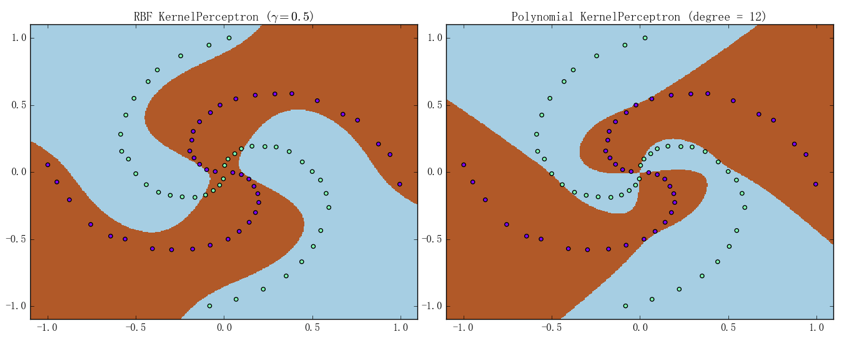左图为 RBF 核感知机（$\gamma = 0.5$）、准确率为 90.0%；右图为多项式核感知机（$p = 12$）、准确率为 98.75%（迭代次数都是$10^{5}$）。虽说效果貌似还不错，但是由它们的训练曲线可以看出、训练过程其实是相当“不稳定”的：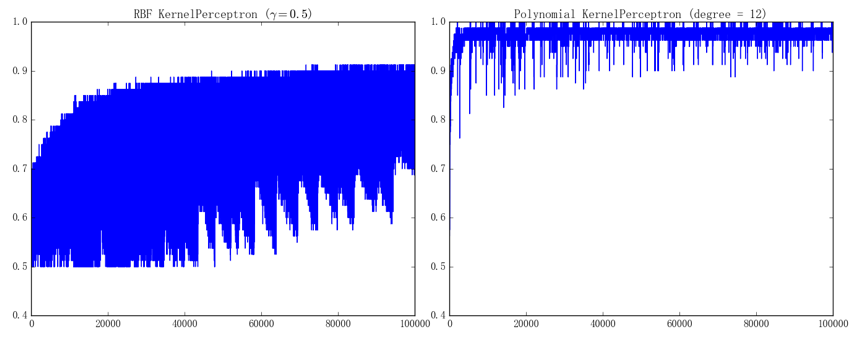左、右图分别对应着 RBF 核感知机和多项式核感知机的训练曲线。之所以有这么大的波动、是因为我们采取的随机梯度下降每次只会进行非常局部的更新，而螺旋线数据集本身又具有比较特殊的结构，从而在直观上也能想象、模型的参数在训练的过程中很容易来回震荡。这一点在 SVM 上也会有体现、因为我们打算实现的 SMO 算法同样也是针对局部（两个变量）进行更新的

# 核 SVM 的实现

接下来就看看核 SVM 的实现，虽说有些繁复、但其实只是一步一步地将之前说过的算法翻译出来而已，如果能理顺算法的逻辑的话、实现本身其实并不困难：

以上就是 SMO 算法中的核心步骤，接下来只需要将它们整合进一个大框架中即可（需要指出的是，随机选取第二个变量虽说效果也不错、但效率终究还是会差上一点；不过考虑到实现的复杂度、我们还是用随机选取的方法来进行实现）：

可以看到大部分代码确实只是算法的直译。同样可以先通过螺旋线数据集来大致看看核 SVM 的分类能力、结果如下图所示（图中用黑圈标注的样本点即是支持向量）：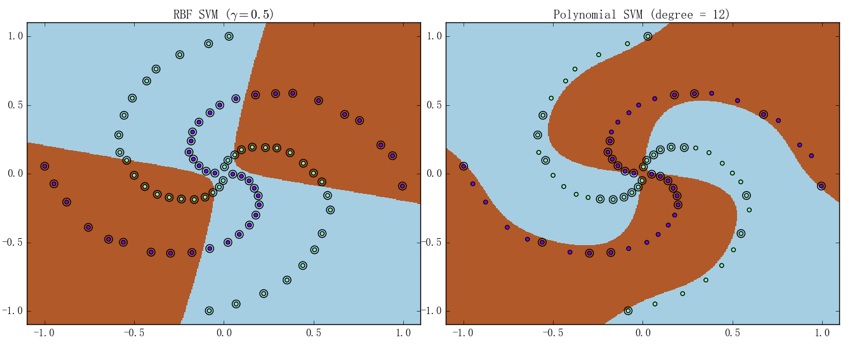左图为 RBF 核 SVM（$\gamma = 0.5$）、迭代了 729 次即达到了停机条件（所有样本的误差都$< \epsilon$）、最终准确率为 51.25%；右图为多项式核 SVM（$p = 12$）、迭代了 6727 次即达到了停机条件、准确率为 97.5%。它们的训练曲线如下图所示：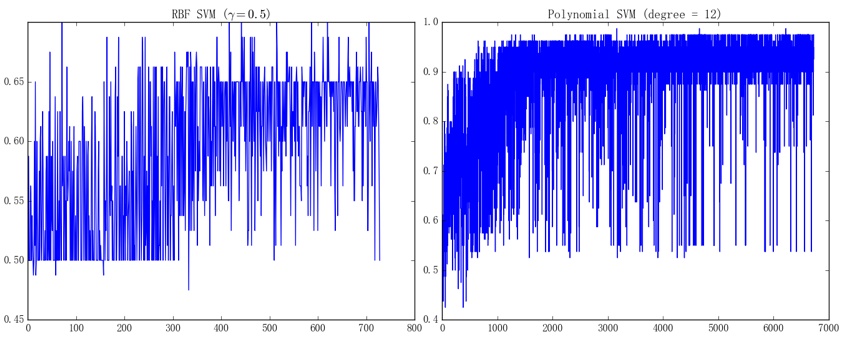左、右图分别对应着 RBF 核 SVM 和多项式核 SVM 的训练曲线。虽说看上去似乎比核感知机的表现还要差、但这毕竟只是一个特殊的情形；事实上、即使是成熟的 SVM 库也并不是万能的。比如如果直接使用螺旋线数据集来训练 sklearn 中的、基于 LibSVM 进行实现的 SVM 模型的话、会得到如下图所示的结果：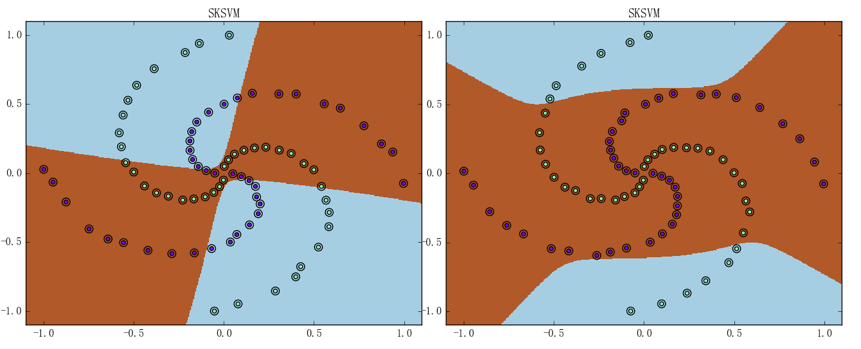左图为 RBF 核 SVM（$\gamma = 0.5$）、最终准确率为 50.0%；右图为多项式核 SVM（$p = 12$）、准确率为 65.0%。造成这种差异的原因在于我们实现的多项式核函数和 sklearn 中的 SVM 所使用的多项式核函数不一样，如果将我们的核函数传进去、是可以得到相似结果的

作为本篇文章的收尾，我们可以通过画出两种核模型在蘑菇数据集上的训练曲线来简单地评估一下模型在真实数据下的表现。为了说明模型的泛化能力，我们只取 100 个样本作为训练样本、并用剩余 8000 多个样本作为测试样本来检验

首先来看一下核感知机的表现：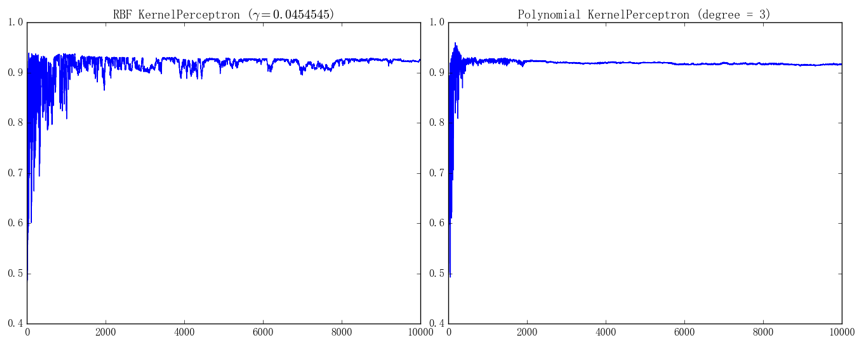左图为 RBF 核感知机（$\gamma \approx 0.04546$）的训练曲线、最终在测试集上的准确率为 92.53%；右图为多项式核感知机（$p = 3$）的训练曲线、最终在测试集上的准确率为 91.59%（迭代次数都是$10^{4}$）。由于只采用了 100 个样本训练、每次训练后的模型表现会波动得比较厉害；不过总体而言、RBF 核感知机会比多项式核感知机波动得更厉害一点

接下来看一下核 SVM 的表现：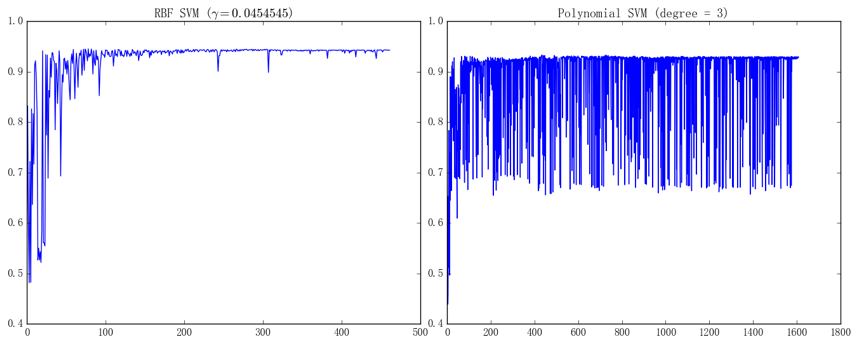左图为 RBF 核 SVM（$\gamma \approx 0.04546$）、迭代了 462 次即达到了停机条件、最终在测试集上的准确率为 94.29%；右图为多项式核 SVM（$p = 3$）、迭代 1609 次即达到了停机条件、最终在测试集上的准确率为 92.96%

]]>
<p>有了上一篇文章的诸多准备、我们就能以之为基础实现核感知机和 SVM 了。不过需要指出的是，由于我们实现的 SVM 是一个朴素的版本、所以如果是要在实际任务中应用 SVM 的话，还是应该使用由前人开发、维护并经过长年考验的成熟的库（比如 LibSVM 等）；这些库能够处理更大的数据和更多的边值情况、运行的速度也会快上很多，这是因为它们通常都使用了底层语言来实现核心算法、且在算法上也做了许多数值稳定性和数值优化的处理</p>
从线性到非线性 http://mlblog.carefree0910.me/posts/924abfe1/ 2017-04-28T03:18:01.000Z 2017-07-04T00:28:54.000Z 前文已经提过，由于对偶形式中的样本点仅以内积的形式出现、所以利用核技巧能将线性算法“升级”为非线性算法。有一个与核技巧（Kernel Trick）类似的概念叫核方法（Kernel Method），这两者的区别可以简单地从字面意思去认知：当我们提及核方法（Method）时、我们比较注重它背后的原理；当我们提及核技巧（Trick）时、我们更注重它实际的应用。考虑到本书的主旨、我们还是选择了核技巧这一说法

注意：以上关于核技巧和核方法这两个名词的区分不是一种共识、而是我个人为了简化问题而作的一种形象的说明，所以切忌将其作为严谨的叙述

# 核技巧简述

虽说重视应用、但一些基本的概念还是需要稍微了解的。核方法本身要深究的话会牵扯到诸如正定核、内积空间、希尔伯特空间乃至于再生核希尔伯特空间（Reproducing Kernel Hilbert Space，常简称为 RKHS）、这些东西又会牵扯到泛函的相关理论，可谓是一个可以单独拿来出书的知识点。幸运的是，单就核技巧而言、我们仅需要知道其中的三个定理即可，这三个定理分别说明了核技巧的合理性、普适性和高效性。不过在叙述这三个定理之前，我们可以先来看看核技巧的直观解释

核技巧往简单地说，就是将一个低维的线性不可分的数据映射到一个高维的空间、并期望映射后的数据在高维空间里是线性可分的。我们以异或数据集为例：在二维空间中、异或数据集是线性不可分的；但是通过将其映射到三维空间、我们可以非常简单地让其在三维空间中变得线性可分。比如定义映射：

该映射的效果如下图所示：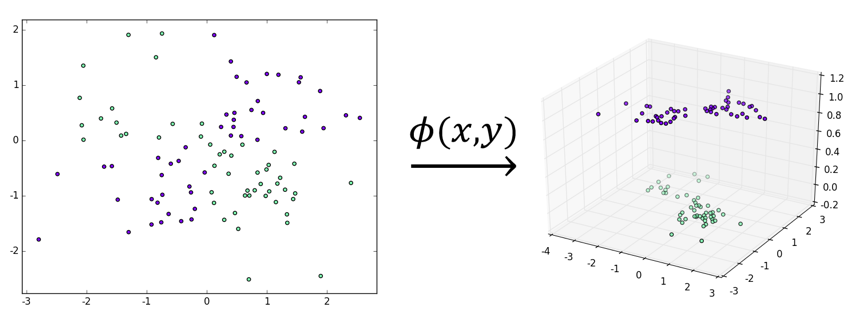可以看到，虽然左图的数据集线性不可分、但显然右图的数据集是线性可分的，这就是核技巧工作原理的一个不太严谨但仍然合理的解释

注意：这里我们暂时采用了“从低维到高维的映射”这一说法、但该说法并不完全严谨，原因会在后文说明、这里只需留一个心眼即可

从直观上来说，确实容易想象、同一份数据在越高维的空间中越有可能线性可分，但从理论上是否确实如此呢？1965 年提出的 Cover 定理解决了这个问题，它的具体叙述如下：若设 d 维空间中 N 个点线性可分的概率为$p(d,N)$，那么就有：

其中

定理的证明细节从略，我们只需要知道它证明了当空间的维数 d 越大时、其中的 N 个点线性可分的概率就越大，这构成了核技巧的理论基础之一

至此，似乎问题就转化为了如何寻找合适的映射$\phi$、使得数据集在被它映射到高维空间后变得线性可分。不过可以想象的是，现实任务中的数据集要比上文我们拿来举例的异或数据集要复杂得多、直接构造一个恰当的$\phi$的难度甚至可能高于解决问题本身。而核技巧的巧妙之处就在于，它能将构造映射这个过程再次进行转化、从而使得问题变得简易：它通过核函数来避免显式定义映射$\phi$。往简单里说、核技巧会通过用核函数

替换各式算法中出现的内积

来完成将数据从低维映射到高维的过程。换句话说、核技巧的思想如下：

• 将算法表述成样本点内积的组合（这经常能通过算法的对偶形式实现）
• 设法找到核函数$K\left( x_i,x_j\right)$，它能返回样本点$x_i$$x_j$$\phi$作用后的内积
• $K\left( x_i,x_j\right)$替换$x_i\cdot x_j$、完成低维到高维的映射（同时也完成了从线性算法到非线性算法的转换）

而核技巧事实上能够应用的场景更为宽泛——在 2002 年由 Sch$\ddot{o}$lkopf 和 Smola 证明的表示定理告诉我们：设$\mathcal{H}$为核函数$K$对应的映射后的空间（RKHS），$\left\| h \right\|_{\mathcal{H}}$表示$\mathcal{H}$$h$的范数，那么对于任意单调递增的函数$C$和任意非负损失函数$L$、优化问题

的解总可以表述为核函数$K$的线性组合

这意味着对于任意一个损失函数和一个单调递增的正则化项组成的优化问题、我们都能够对其应用核技巧。所以至此、大多数的问题就转化为如何找到能够表示成高维空间中内积的核函数了。幸运的是、1909 年提出的 Mercer 定理解决了这个问题，它的具体叙述如下：$K\left( x_{i},x_{j} \right)$若满足

亦即如果$K\left( x_{i},x_{j} \right)$是对称函数的话、那么它具有 Hilbert 空间中内积形式的充要条件有以下两个：

• 对任何平方可积$g$、满足
• 对含任意 N 个样本的数据集$D=\left\{ x_1,...,x_N\right\}$、核矩阵： 是半正定矩阵

注意：通常我们会称满足这两个充要条件之一的函数为 Mercer 核函数而把核函数定义得更宽泛。由于本书不打算在理论上深入太多、所以一律将 Mercer 核函数简称为核函数。此外，虽说 Mercer 核函数确实具有 Hilbert 空间中的内积形式、但此时的 Hilbert 空间并不一定具有“维度”这么好的概念（或说、可以认为此时 Hilbert 空间的维度为无穷大，比如下面马上就要讲到的 RBF 核、它映射后的空间就是无穷维的）。这也正是为何前文说“从低维到高维的映射”不完全严谨

Mercer 定理为寻找核函数带来了极大的便利。可以证明如下两族函数都是核函数：

• 多项式核

# 核技巧的应用

我们接下来会实现的也正是这两族核函数对应的、应用了核技巧的算法，具体而言、我们会利用核技巧来将感知机和支持向量机算法从原始的线性版本“升级”为非线性版本

由简入繁、先从核感知机讲起；由于感知机对偶算法十分简单、对其应用核技巧相应的也非常平凡——直接用核函数替换掉相应内积即可。不过需要注意的是，由于我们采用的是随机梯度下降、所以算法中也应尽量只更新局部参数以避免进行无用的计算：

1. 输入：训练数据集$D = \{\left( x_{1},y_{1} \right),\ldots,\left( x_{N},y_{N} \right)\}$、迭代次数 M、学习速率$\eta$，其中：
2. 过程

1. 初始化参数：

同时计算核矩阵：

2. $j = 1,\ldots,M$

1. $E = \varnothing$（亦即没有误分类的样本点）则退出循环体
2. 否则，任取$E$中的一个样本点$(x_{i},y_{i})$并利用其下标更新局部参数：
3. 利用$dw$$db$更新预测向量$\hat{y}$其中、$\mathbf{K}_{i\mathbf{\cdot}}$表示$\mathbf{K}$的第 i 行、$\mathbf{1}$表示全为 1 的向量
3. 输出：感知机模型$g\left( x \right) = \text{sign}\left( f\left( x \right) \right) = sign\left( \sum_{i = 1}^{N}{\alpha_{i}y_{i}\left( K\left( x_{i},x \right) + 1 \right)} \right)$

再来看如何对 SVM 应用核技巧。虽说在对偶算法上应用核技巧是非常自然、直观的，但是直接在原始算法上应用核技巧也无不可

注意原始问题可以表述为：

若令$w = \sum_{i = 1}^{N}{u_{i}\phi(x_{i})} = u \cdot \phi(x)$、其中：

则可知上述问题能够通过$\phi$映射到高维空间上：

亦即

利用一定的技巧是可以直接利用梯度下降法直接对这个无约束最优化问题求解的，不过相关的数学理论基础都相当繁复、实现起来也有些麻烦；尽管如此、还是有许多优秀的算法是基于上述思想的

直观起见、我们还是将重点放在如何对 SMO 应用核技巧的讨论上。由于前文已经说明了 SMO 的大致步骤，所以我们先补充说明当时没有讲到的、选出两个变量后应该如何继续求解，然后再来看具体的算法应该如何叙述

使得对$i = 1,\ldots,N$、都有

不妨设$i=1$$j=2$，那么在针对$\alpha_1$$\alpha_2$的情况下，$\alpha_3,...,\alpha_N$是固定的、且上述最优化问题可以转化为：

使得对$i = 1$$i = 2$、有

其中$const$为常数。可以看出此时问题确实转化为了一个带约束的二次函数求极值问题、从而能够比较简单地求出其解析解。推导过程从略、以下就直接在算法中写出结果：

1. 输入：训练数据集$D = \{\left( x_{1},y_{1} \right),\ldots,\left( x_{N},y_{N} \right)\}$、迭代次数 M、容许误差$\epsilon$，其中：
2. 过程

1. 初始化参数： 同时计算核矩阵：
2. $j = 1,\ldots,M$

1. 选出违反 KKT 条件最严重的样本点$(x_{i},y_{i})$，若其违反程度小于$\epsilon$、则退出循环体
2. 否则、选出异于i的任一个下标 j，针对$\alpha_{i}$$\alpha_{j}$构造一个新的只有两个变量二次规划问题并求出解析解。具体而言，首先要更新的是$\alpha_{2}$、它由以下几个参数定出：

考虑到约束条件、我们需要定出新的$\alpha_{2}$下上界：

继而根据$l$$h$$\alpha_{2}^{new,raw}$进行“裁剪”即可：

这里要注意记录$\alpha_{2}$的增量：

3. 利用$\Delta\alpha_{2}$更新$\alpha_{1}$、同时注意记录$\alpha_{1}$的增量：
4. 利用$\Delta\alpha_{1}$$\Delta\alpha_{2}$进行局部更新： 其中
5. 利用$dw$$db$更新预测向量$\hat{y}$其中、$\mathbf{K}_{\mathbf{i}\mathbf{\cdot}}$表示$\mathbf{K}$的第i行、$\mathbf{1}$表示全为1的向量
3. 输出：感知机模型$g\left( x \right) = \text{sign}\left( f\left( x \right) \right) = sign\left( \sum_{i = 1}^{N}{\alpha_{i}y_{i}K\left( x_{i},x \right)} + b \right)$、其中：

这里的下标 k 满足

可以用反证法证明这样的下标 k 必存在、具体步骤从略

从这两种算法应用核技巧的方式可以看出，虽然它们应用的训练算法完全不同（一个是随机梯度下降、一个是序列最小最优化）、但它们每一次迭代中做的事情却有相当多是一致的；为了合理重复利用代码、我们可以先把对应的实现都抽象出来：

其中定义 RBF 核函数时用到了升维的操作、这算是 Numpy 的高级使用技巧之一；具体的思想和机制会在后续的文章中进行简要说明、这里就暂时按下不表

以上我们就搭好了基本的框架、接下来要做的就是继续把具有普适性的训练过程进行抽象和实现：

注意到我们调用了一个叫KernelConfig的类、它的定义很简单：

亦即默认惩罚因子$C$为 1、多项式核的次数$p$为 3。同时需要注意的是，我们在循环体里面调用了_fit核心方法、在最后调用了_update_params方法，这两个方法都是留给子类定义的；不过比较巧妙的是，无论是记录$\hat{y}$_prediction_cache的更新还是预测函数predict`的定义、都可以写成同一种形式：

]]>
<p>前文已经提过，由于对偶形式中的样本点仅以内积的形式出现、所以利用核技巧能将线性算法“升级”为非线性算法。有一个与核技巧（Kernel Trick）类似的概念叫核方法（Kernel Method），这两者的区别可以简单地从字面意思去认知：当我们提及核方法（Method）时、我们比较注重它背后的原理；当我们提及核技巧（Trick）时、我们更注重它实际的应用。考虑到本书的主旨、我们还是选择了核技巧这一说法</p> <p><strong><em>注意：以上关于核技巧和核方法这两个名词的区分不是一种共识、而是我个人为了简化问题而作的一种形象的说明，所以切忌将其作为严谨的叙述</em></strong></p>